# Data visualisation

This past week, I spent two days learning the basics of representing data in Tableau, which led to a discussion about Stephen Few, who has some opinions about clearly representing your data.

That, in turn, got me thinking about one of my old hobby horses – my personal mission to eliminate the world of spider plots.

# Acoustic memory

This video shows the inner workings of an early electronic calculator. The cool thing is that it uses a delay-line memory based on an acoustic signal running down a length of steel.

# DFT’s Part 2: It’s a little complex…

## Whole Numbers and Integers

Once upon a time you learned how to count. You were probably taught to count your fingers… 1, 2, 3, 4 and so on. Although no one told you so at the time, you were being taught a set of numbers called whole numbers.

Sometime after that, you were probably taught that there’s one number that gets tacked on before the ones you already knew – the number 0.

A little later, sometime after you learned about money and the fact that we don’t have enough, you were taught negative numbers… -1, -2, -3 and so on. These are the numbers that are less than 0.

That collection of numbers is called integers – all “countable” numbers that are negative, zero and positive. So the collection is typically written

… -5, -4, -3, -2, -1, 0, 1, 2, 3, 4, 5 …

## Rational Numbers

Eventually, after you learned about counting and numbers, you were taught how to divide (the mathematical word for “sharing equally”). When someone said “20 divided by 5 equals 4” then they meant “if you have 20 sticks, then you could put those sticks in 4 piles with 5 sticks in each pile.” Eventually, you learned that the division of one number by another can be written as a fraction like 3/1 or 20/5 or 5/4 or 1/3.

If you do that division the old-fashioned way, you get numbers like this:

3 1 = 3.000000000 etc…

20 5 = 4.00000000 etc…

5 4 = 1.200000000 etc…

1 3 = 0.333333333 etc…

The thing that I’m trying to point out here is that eventually, these numbers start repeating sometime after the decimal point. These numbers are called rational numbers.

## Irrational Numbers

What happens if you have a number that doesn’t start repeating, no matter how many numbers you have? Take a number like the square root of 2 for example. This is a number that, when you multiply it by itself, results in the number 2. This number is approximately 1.4142. But, if we multiply 1.4142 by 1.4142, we get 1.99996164 – so 1.4142 isn’t exactly the square root of 2. In fact, if we started calculating the exact square root of 2, we’d result in a number that keeps going forever after the decimal place and never repeats. Numbers like this (π is another one…) that never repeat after the decimal are called irrational numbers

## Real Numbers

All of these number types – rational numbers (which includes integers) and irrational numbers fall under the general heading of real numbers. The fact that these are called “real” implies immediately that there is a classification of numbers that are “unreal” – but we’ll get to that later…

## Imaginary Numbers

Let’s think about the idea of a square root. The square root of a number is another number which, when multiplied by itself is the first number. For example, 3 is the square root of 9 because 3*3 = 9. Let’s consider this a little further: a positive number muliplied by itself is a positive number (for example, 4*4 = 16… 4 is positive and 16 is also positive). A negative number multiplied by itself is also positive (i.e. 4*-4 = 16).

Now, in the first case, the square root of 16 is 4 because 4*4 = 16. (Some people would be really picky and they’ll tell you that 16 has two roots: 4 and -4. Those people are slightly geeky, but technically correct.) There’s just one small snag – what if you were asked for the square root of a negative number? There is no such thing as a number which, when multiplied by itself results in a negative number. So asking for the square root of -16 doesn’t make sense. In fact, if you try to do this on your calculator, it’ll probably tell you that it gets an error instead of producing an answer.

For a long time, mathematicians just called the square root of a negative number “imaginary” since it didn’t exist – like an imaginary friend that you had when you were 2… However, mathematicians as a general rule don’t like loose ends – they aren’t the type of people who leave things lying around… and having something as simple as the square root of a negative number lying around unanswered got on their nerves.

Then, in 1797, a Norwegian surveyor named Casper Wessel presented a paper to the Royal Academy of Denmark that described a new idea of his. He started by taking a number line that contains all the real numbers like this:

He then pointed out that multiplying a number by -1 was the same as rotating by an angle of 180º, like this:

He then reasoned that, if this were true, then the square root of -1 must be the same as rotating by 90º.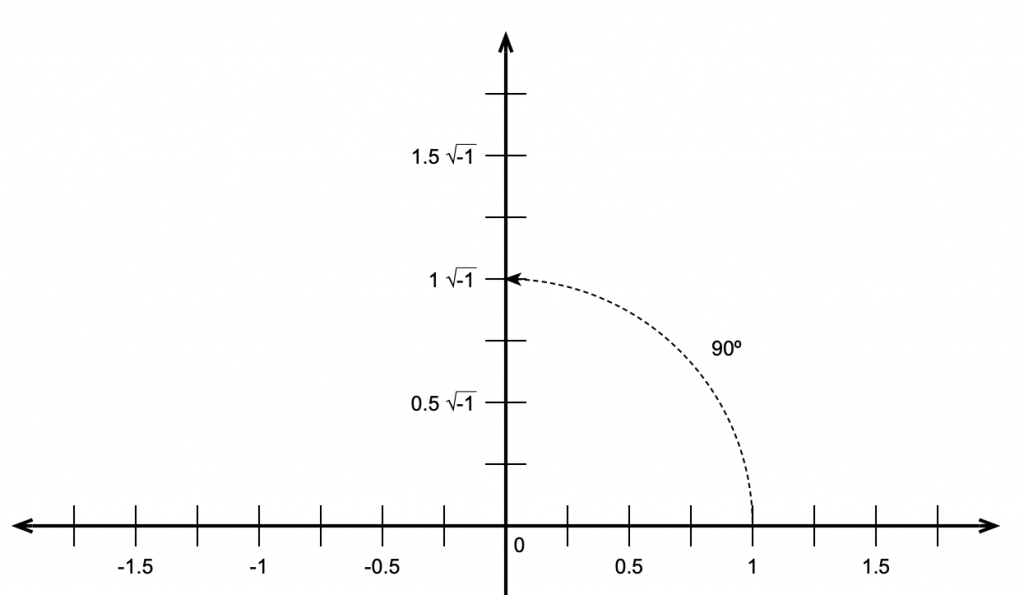Figure 3: This means that the square root of -1 must be the same a rotating by 90º.

This meant that the number line we started with containing the real numbers is the X-axis on a 2-dimensional plane where the Y-axis contains the imaginary numbers. That plane is called the Z plane, where any point (which we’ll call ‘Z’) is the combination of a real number (X) and an imaginary number (Y).

If you look carefully at Figure 4, you’ll see that I used a “j” to indicate the imaginary portion of the number. Generally speaking, mathematicians use and physicists and engineers use so we’ll stick with j. (The reason physics and engineering people use j is that they use i to mean “electrical current”.)

“What is j?” I hear you cry. Well, is just the square root of -1. Of course, there is no number that is the square root of -1

and therefore

Now, remember that = -1. This is useful for any square root of any negative number, you just calculate the square root of the number pretending that it was positive, and then stick a after it. So, since the square root of 16, abbreviated sqrt(16) = 4 and sqrt(-1) = j, then sqrt(-16) = 4j.

## Complex numbers

Now that we have real and imaginary numbers, we can combine them to create a complex number. Remember that you can’t just mix real numbers with imaginary ones – you keep them separate most of the time, so you see numbers like

3+2j

This is an example of a complex number that contains a real component (the 3) and an imaginary component (the 2j). In some cases, these numbers are further abbreviated with a single Greek character, like α or β, so you’ll see things like

α = 3+2j

In other cases, you’ll see a bold letter like the following:

Z = 3+2j

A lot of people do this because they like reserving Greek letters like α and ϕ for variables associated with angles.

Personally, I like seeing the whole thing – the real and the imaginary components – no reducing them to single Greek letters (they’re for angles!) or bold letters.

## Absolute Value (aka the Modulus)

The absolute value of a complex number is a little weirder than what we usually think of as an absolute value. In order to understand this, we have to look at complex numbers a little differently:

Remember that j*j = -1.

Also, remember that, if we have a cosine wave and we delay it by 90º and then delay it by another 90º, it’s the same as inverting the polarity of the cosine, in other words, multiplying the cosine by -1. So, we can think of the imaginary component of a complex number as being a real number that’s been rotated by 90º, we can picture it as is shown in the figure below.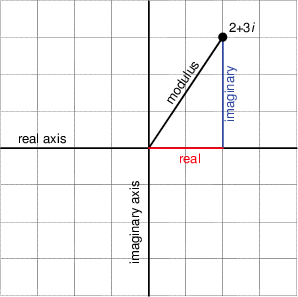Figure 5. The relationship bewteen the real and imaginary components for the number (2 + 3 j). Notice that the X and Y axes have been labeled the “real” and “imaginary” axes.

Notice that Figure 5 actually winds up showing three things. It shows the real component along the x-axis, the imaginary component along the y-axis, and the absolute value or modulus of the complex number as the hypotenuse of the triangle. This is shown in mathematical notation in exactly the same way as in normal math – with vertical lines. For example, the modulus of 2+3j is written |2+3j|

This should make the calculation for determining the modulus of the complex number almost obvious. Since it’s the length of the hypotenuse of the right triangle formed by the real and imaginary components, and since we already know the Pythagorean theorem then the modulus of the complex number (a + b j) is

Given the values of the real and imaginary components, we can also calculate the angle of the hypotenuse from horizontal using the equation

This will come in handy later.

## Complex notation or… Who cares?

This is probably the most important question for us. Imaginary numbers are great for mathematicians who like wrapping up loose ends that are incurred when a student asks “what’s the square root of -1?” but what use are complex numbers for people in audio? Well, it turns out that they’re used all the time, by the people doing analog electronics as well as the people working on digital signal processing. We’ll get into how they apply to each specific field in a little more detail once we know what we’re talking about, but let’s do a little right now to get a taste.

In the previous posting, that introduces the trigonometric functions sine and cosine, we looked at how both functions are just one-dimensional representations of a two-dimensional rotation of a wheel. Essentially, the cosine is the horizontal displacement of a point on the wheel as it rotates. The sine is the vertical displacement of the same point at the same time. Also, if we know either one of these two components, we know:

1. the diameter of the wheel and
2. how fast it’s rotating

but we need to know both components to know the direction of rotation.

At any given moment in time, if we froze the wheel, we’d have some contribution of these two components – a cosine component and a sine component for a given angle of rotation. Since these two components are effectively identical functions that are 90º apart (for example, a cossine wave is the same as a sine that’s been delayed by 90º) and since we’re thinking of the real and imaginary components in a complex number as being 90º apart, then we can use complex math to describe the contributions of the sine and cosine components to a signal.

Huh?

Let’s look at an example. If the signal we wanted to look at a signal that consisted only of a cosine wave, then we’d know that the signal had 100cosine and 0sine. So, if we express the cosine component as the real component and the sine as the imaginary, then what we have is:

1 + 0 j

If the signal were an upside-down cosine, then the complex notation for it would be (1 + 0 jbecause it would essentially be a cosine * -1 and no sine component. Similarly, if the signal was a sine wave, it would be notated as (0 1 j).

This last statement should raise at least one eyebrow… Why is the complex notation for a positive sine wave (0 1 j)? In other words, why is there a negative sign there to represent a positive sine component? (Hint – we want the wheel to turn clockwise… and clocks turn clockwise to maintain backwards compatibility with an earlier technology – the sundial. So, we use a negative number because of the direction of rotation of the earth…)

This is fine, but what if the signal looks like a sinusoidal wave that’s been delayed a little? As we saw in the previous posting, we can create a sinusoid of any delay by adding the cosine and sine components with appropriate gains applied to each.

So, is we made a signal that were 70.7sine and 70.7cosine. (If you don’t know how I arrived that those numbers, check out the previous posting.) How would you express this using complex notation? Well, you just look at the relative contributions of the two components as before:

0.707 – 0.707 j

It’s interesting to notice that, although this is actually a combination of a cosine and a sine with a specific ratio of amplitudes (in this case, both at 0.707 of “normal”), the result will look like a sine wave that’s been shifted in phase by -45º (or a cosine that’s been phase-shifted by 45º). In fact, this is the case – any phase-shifted sine wave can be expressed as the combination of its sine and cosine components with a specific amplitude relationship.

Therefore (again), any sinusoidal waveform with any phase can be simplified and expressed as its two elemental components, the gains applied to the cosine (or real) and the sine (or imaginary). Once the signal is broken into these two constituent components, it cannot be further simplified.

Link to DFT’s Part 3: The Math

# Fc ≠ Fc

I was working on the sound design of a loudspeaker last week with some new people and software – so we had to get some definitions straight before we messed things up by thinking that we were using the same words to mean the same thing. I’ve made a similar mistake to this before, as I’ve written about here – and I don’t being reminded of my own stupidity repeatedly… (Or, as Stephen Wright once said “I’m having amnesia and deja vu at the same time – I think I’ve forgotten this before…”)

So, in this case on that day, we were talking about the lowly 2nd-order Low Pass Filter, based on a single biquad.

If you read about how to find the cutoff frequency of a low-pass filter, you’ll probably find out that you find the frequency where the gain is one half of the power of that in the bandpass portion of the filter’s response. Since 10*log10(0.5) = -3.01 dB, then this is also called the “3 dB down point” of the filter.

In my case, when I’m implementing a filter, I use the math provided by Robert Bristow-Johnson to calculate my biquad coefficients. You input a cutoff frequency (Fc), and a Q value, and (for a given sampling rate) you get your biquad coefficients.

The question then, is: is the desired cutoff frequency the actual measurable cutoff frequency of the system? (Let’s assume for the purposes of this discussion that there are no other components in the system that affect the magnitude response – just to keep it simple.)

For example, if I make a 2nd-order low pass filter with a desired cutoff frequency of 1 kHz (using a high enough sampling rate to not introduce any errors due to the bilinear transform) and I vary the Q from something very small (in this example, 0.1) to something pretty big (in this example, 20) I get magnitude response curves that look like the figure below.

It is probably already evident that these 25 filter responses plotted above that they do not all cross each other at the 1 kHz line. In addition, you may notice that there is only one of those curves that is -3.01 dB at 1 kHz – when the Q = 1/sqrt(2) or 0.707.

This begs the question: what is the gain of each of those filters at the desired value of Fc (in this case, 1 kHz)? This is plotted as the red line in the figure below.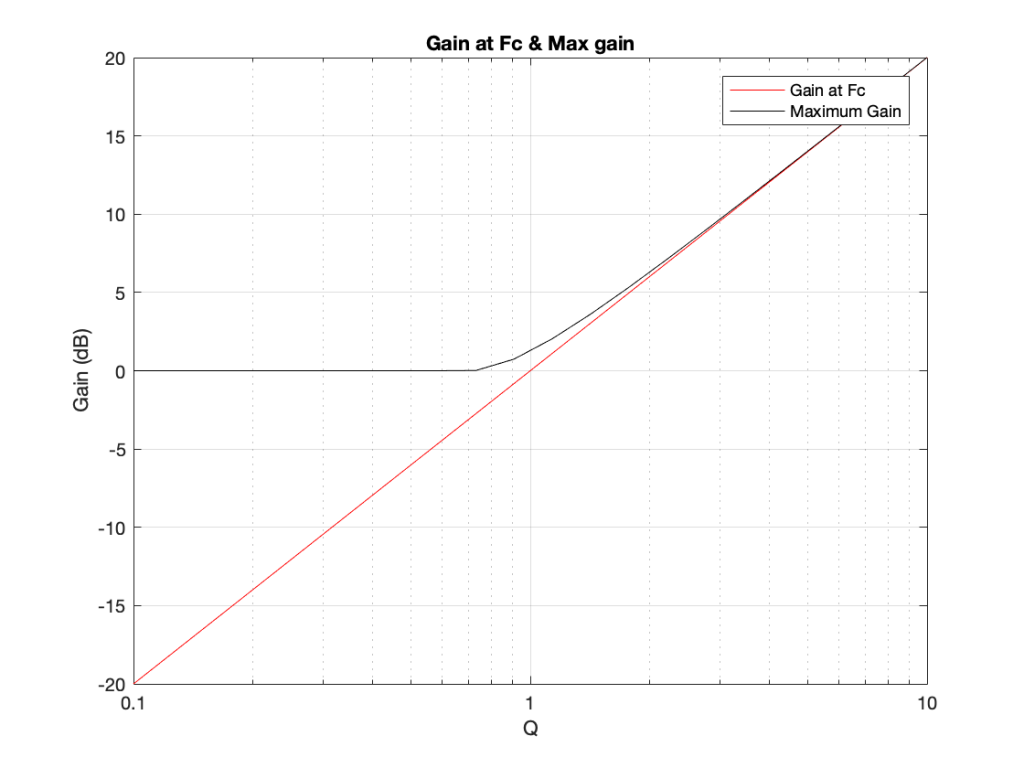The actual gain value of the filters at the desired Fc, and the maximum gain at any frequency.

This plot also shows the maximum gain of the filters for different values of Q. Notice that, in the low end, the maximum value is 0 dB, since the low pass filters only roll off. However, for Q values higher than 1/sqrt(2), there is an overshoot in the response, resulting in a boost at some frequency. As the Q increases, the frequency at which the gain of the filter is highest approaches the desired cutoff frequency. (As can be seen in the plot above, by the time you get to a Q of 20, the gain at Fc and the maximum gain of the filter are the same.)

It may be intuitively interesting (or interestingly intuitive) to note that, when Q goes to infinity, the gain at Fc also goes to infinity, and (relatively speaking) all other frequencies are infinitely attenuated – so you have a sine wave generator.

So, we know that the gain value at the stated Fc is not -3 dB for all but one value of Q. So, what is the -3 dB point, if we state a desired Fc of 1 kHz and we vary the Q? This is shown in the figure below.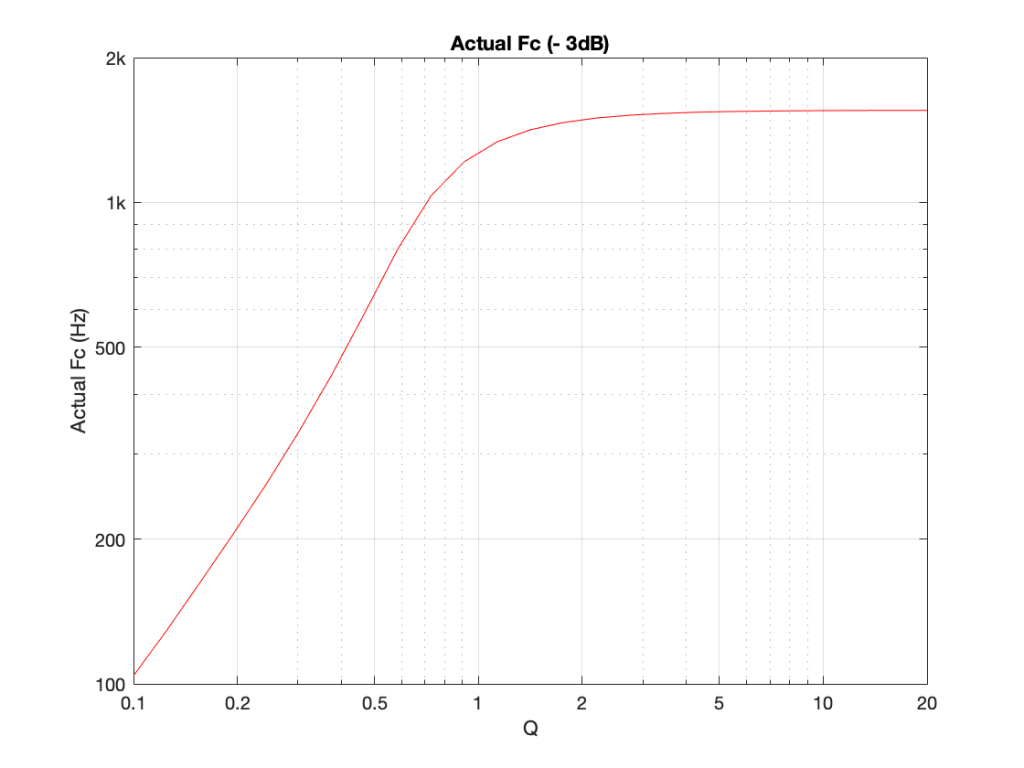The -3 dB point of a 2nd order 1 kHz low pass filter as a function of Q.

So, varying the Q from 0.1 to 20 varies the actual Fc (or, at least, the -3 dB point) from about 104 Hz to about 1554 Hz.

Or, if we plot the same information as a function (or just a multiple) of the desired Fc, you get the plot below.

So, if you’re sitting in a meeting, and the person in front of you is looking at a measurement of a loudspeaker magnitude response, and they say “could you please put in a low pass filter with a cutoff frequency of 1 kHz and a Q of 0.5” you should start asking questions by what, exactly, they mean by “cutoff frequency”… If not, you might just wind up with nice-looking numbers but strangely-sounding loudspeakers.

# Typical Errors in Digital Audio: Part 3 – Aliasing

Reminder: This is still just the lead-up to the real topic of this series. However, we have to get some basics out of the way first…

In the first posting in this series, I talked about digital audio (more accurately, Linear Pulse Code Modulation or LPCM digital audio) is basically just a string of stored measurements of the electrical voltage that is analogous to the audio signal, which is a change in pressure over time… In the second posting in the series, we looked at a “trick” for dealing with the issue of quantisation (the fact that we have a limited resolution for measuring the amplitude of the audio signal). This trick is to add dither (a fancy word for “noise”) to the signal before we quantise it in order to randomise the error and turn it into noise instead of distortion.

In this posting, we’ll look at some of the problems incurred by the way we carve up time into discrete moments when we grab those samples.

Let’s make a wheel that has one spoke. We’ll rotate it at some speed, and make a film of it turning. We can define the rotational speed in RPM – rotations per minute, but this is not very useful. In this case, what’s more useful is to measure the wheel rotation speed in degrees per frame of the film.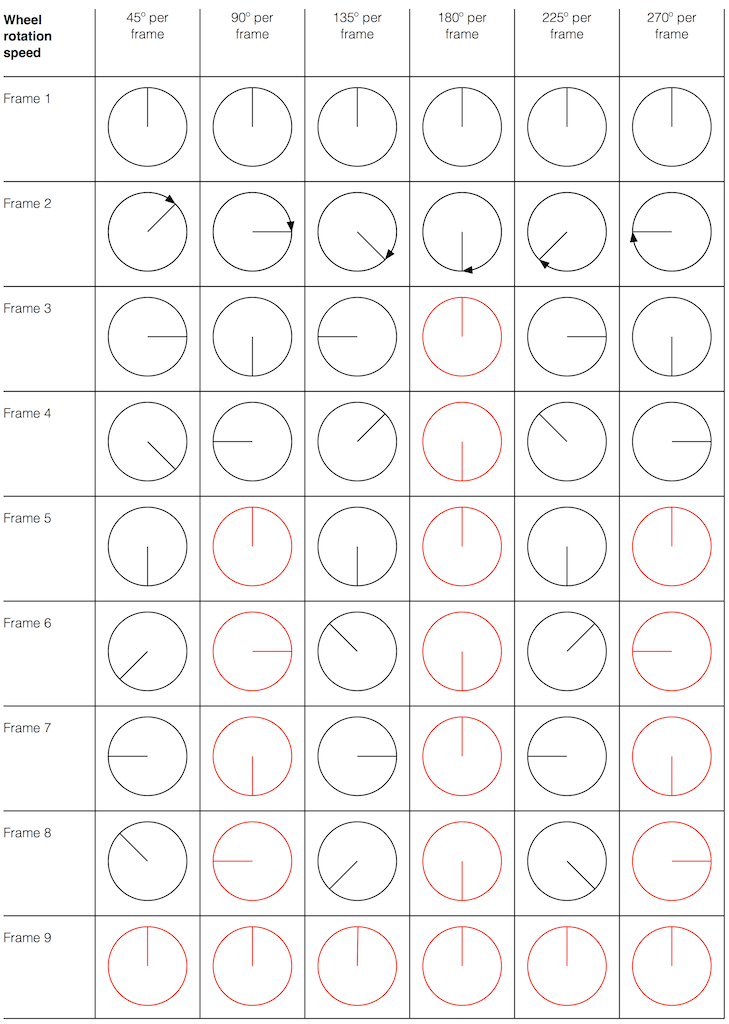Fig 1. The position of a clockwise-rotating wheel (with only one spoke) for 9 frames of a film. Each column shows a different rotational speed of the wheel. The far left column is the slowest rate of rotation. The far right column is the fastest rate of rotation. Red wheels show the frame in which the sequence starts repeating.

Take a look at the left-most column in Figure 1. This shows the wheel rotating 45º each frame. If we play back these frames, the wheel will look like it’s rotating 45º per frame. So, the playback of the wheel rotating looks the same as it does in real life.

This is more or less the same for the next two columns, showing rotational speeds of 90º and 135º per frame.

However, things change dramatically when we look at the next column – the wheel rotating at 180º per frame. Think about what this would look like if we played this movie (assuming that the frame rate is pretty fast – fast enough that we don’t see things blinking…) Instead of seeing a rotating wheel with only one spoke, we would see a wheel that’s not rotating – and with two spokes.

This is important, so let’s think about this some more. This means that, because we are cutting time into discrete moments (each frame is a “slice” of time) and at a regular rate (I’m assuming here that the frame rate of the film does not vary), then the movement of the wheel is recorded (since our 1 spoke turns into 2) but the direction of movement does not. (We don’t know whether the wheel is rotating clockwise or counter-clockwise. Both directions of rotation would result in the same film…)

Now, let’s move over one more column – where the wheel is rotating at 225º per frame. In this case, if we look at the film, it appears that the wheel is back to having only one spoke again – but it will appear to be rotating backwards at a rate of 135º per frame. So, although the wheel is rotating clockwise, the film shows it rotating counter-clockwise at a different (slower) speed. This is an effect that you’ve probably seen many times in films and on TV. What may come as a surprise is that this never happens in “real life” unless you’re in a place where the lights are flickering at a constant rate (as in the case of fluorescent or some LED lights, for example).

Again, we have to consider the fact that if the wheel actually were rotating counter-clockwise at 135º per frame, we would get exactly the same thing on the frames of the film as when the wheel if rotating clockwise at 225º per frame. These two events in real life will result in identical photos in the film. This is important – so if it didn’t make sense, read it again.

This means that, if all you know is what’s on the film, you cannot determine whether the wheel was going clockwise at 225º per frame, or counter-clockwise at 135º per frame. Both of these conclusions are valid interpretations of the “data” (the film). (Of course, there are more – the wheel could have rotated clockwise by 360º+225º = 585º or counter-clockwise by 360º+135º = 495º, for example…)

Since these two interpretations of reality are equally valid, we call the one we know is wrong an alias of the correct answer. If I say “The Big Apple”, most people will know that this is the same as saying “New York City” – it’s an alias that can be interpreted to mean the same thing.

We people in audio commit many sins. One of them is that, every time we draw a plot of anything called “audio” we start out by drawing a sine wave. (A similar sin is committed by musicians who, at the first opportunity to play a grand piano, will play a middle-C, as if there were other notes in the world.) The question is: what, exactly, is a sine wave?

Get a Slinky – or if you don’t want to spend money on a brand name, get a spring. Look at it from one end, and you’ll see that it’s a circle, as can be (sort of) seen in Figure 2.

Since this is a circle, we can put marks on the Slinky at various amounts of rotation, as in Figure 3.

Of course, I could have put the 0º marl anywhere. I could have also rotated counter-clockwise instead of clockwise. But since both of these are arbitrary choices, I’m not going to debate either one.

Now, let’s rotate the Slinky so that we’re looking at from the side. We’ll stretch it out a little too…

Let’s do that some more…

When you do this, and you look at the Slinky directly from one side, you are able to see the vertical change of the spring from the centre as a result of the change in rotation. For example, we can see in Figure 6 that, if you mark the 45º rotation point in this view, the distance from the centre of the spring is 71% of the maximum height of the spring (at 90º).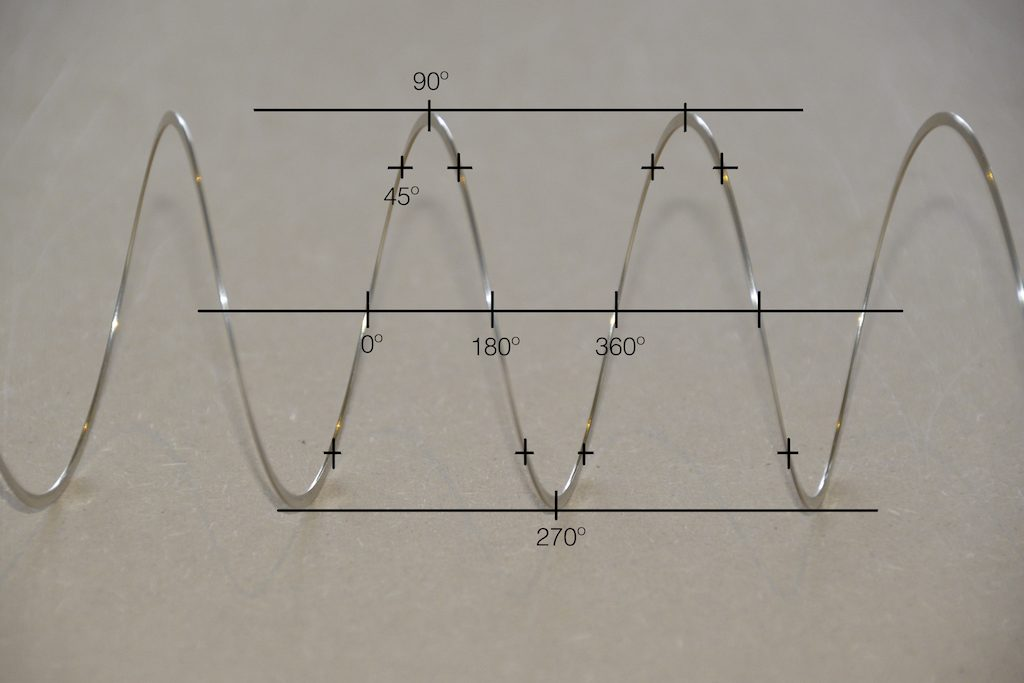Fig 6. The same markings shown in Figure 3, when looking at the Slinky from the side. Note that, if we didn’t have the advantage of a little perspective (and a spring made of flat metal), we would not know whether the 0º point was closer or further away from us than the 180º point. In other words, we wouldn’t know if the Slinky was rotating clockwise or counter-clockwise.

So what? Well, basically, the “punch line” here is that a sine wave is actually a “side view” of a rotation. So, Figure 7, shows a measurement – a capture – of the amplitude of the signal every 45º.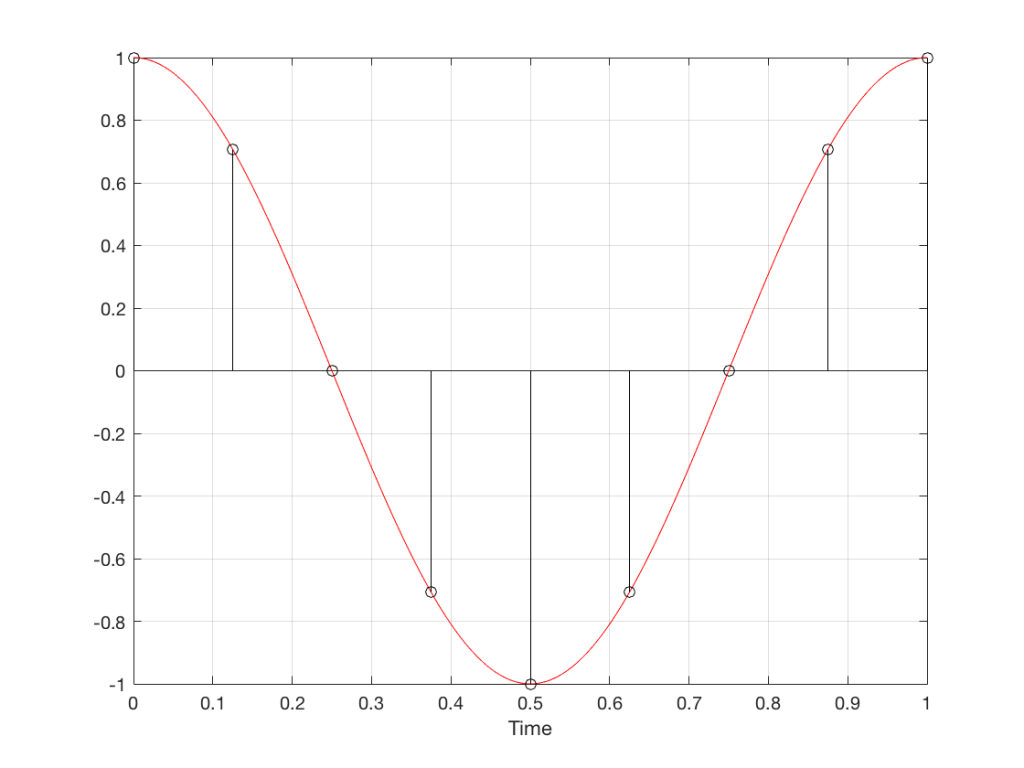Fig 7. Each measurement (a black “lollipop”) is a measurement of the vertical change of the signal as a result of rotating 45º.

Since we can now think of a sine wave as a rotation of a circle viewed from the side, it should be just a small leap to see that Figure 7 and the left-most column of Figure 1 are basically identical.

Let’s make audio equivalents of the different columns in Figure 1.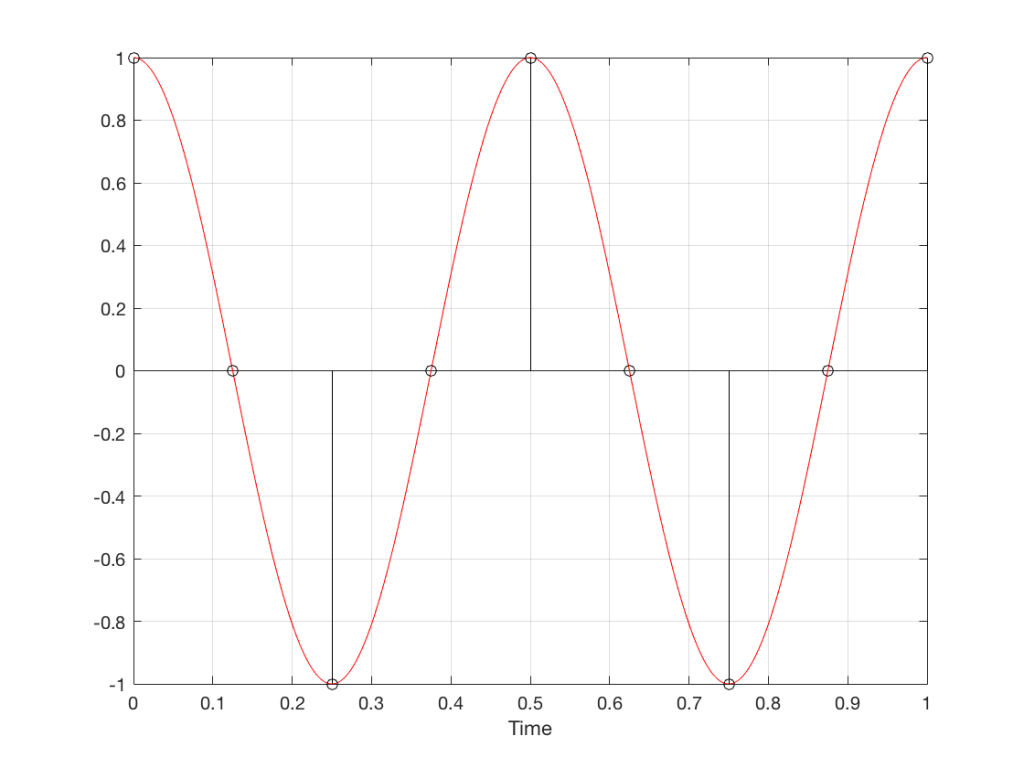Fig 8. A sampled cosine wave where the frequency of the signal is equivalent to 90º per sample period. This is identical to the “90º per frame” column in Figure 1.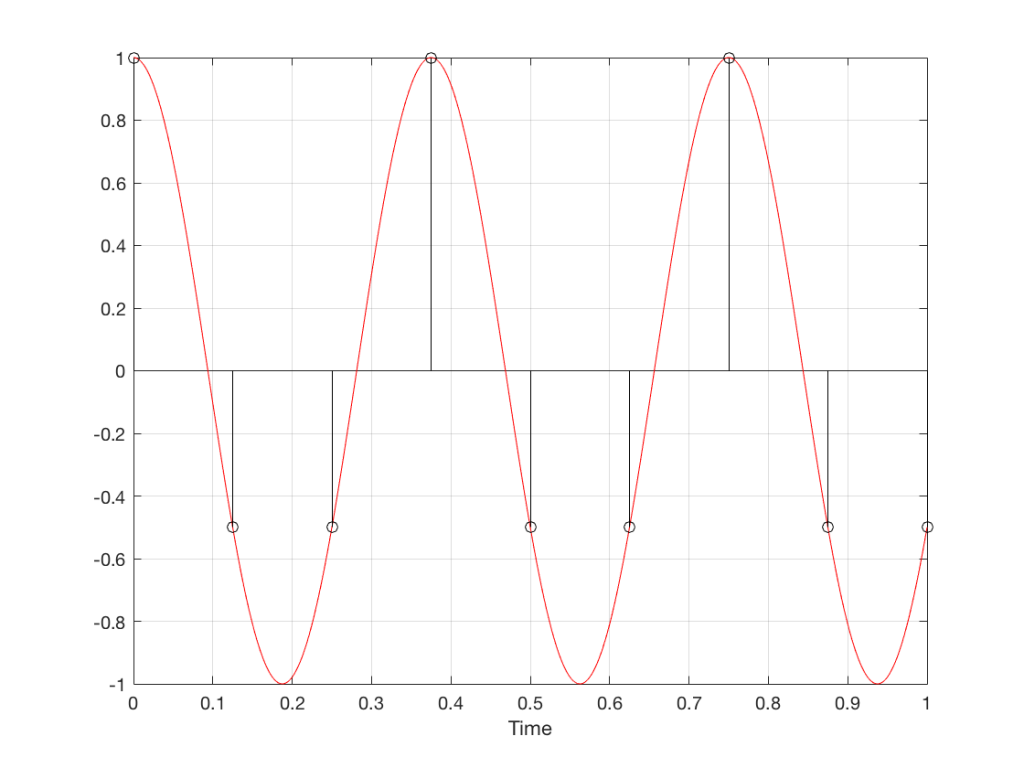Fig 9. A sampled cosine wave where the frequency of the signal is equivalent to 135º per sample period. This is identical to the “135º per frame” column in Figure 1.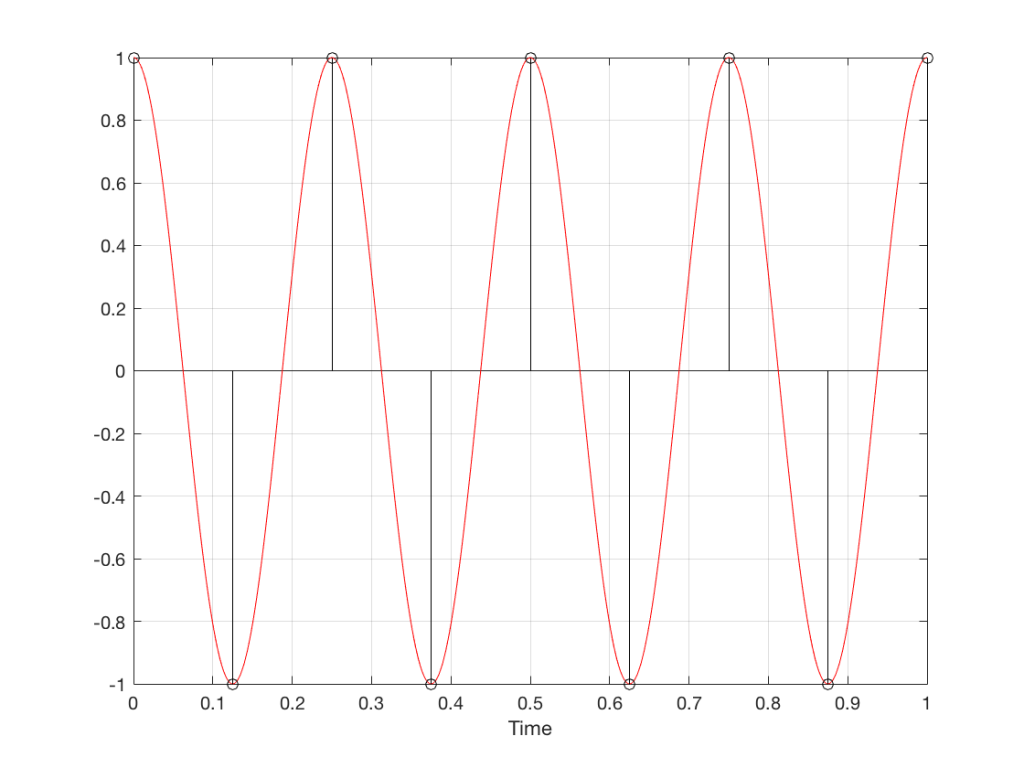Fig 10. A sampled cosine wave where the frequency of the signal is equivalent to 180º per sample period. This is identical to the “180º per frame” column in Figure 1.

Figure 10 is an important one. Notice that we have a case here where there are exactly 2 samples per period of the cosine wave. This means that our sampling frequency (the number of samples we make per second) is exactly one-half of the frequency of the signal. If the signal gets any higher in frequency than this, then we will be making fewer than 2 samples per period. And, as we saw in Figure 1, this is where things start to go haywire.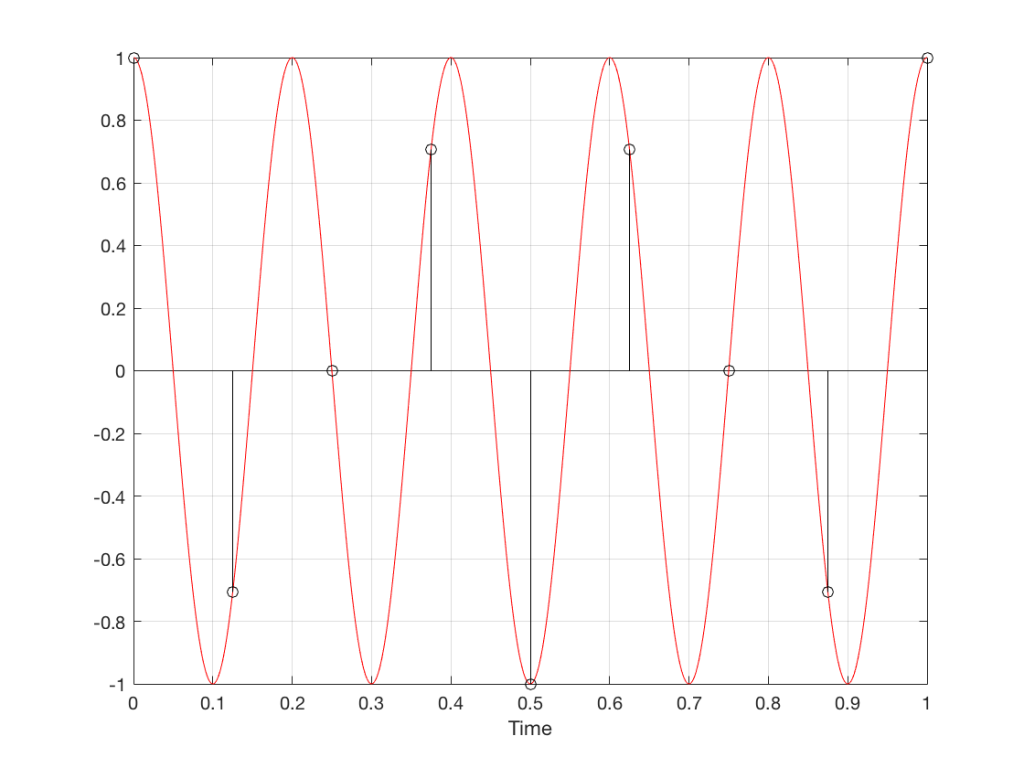Fig 11. A sampled cosine wave where the frequency of the signal is equivalent to 225º per sample period. This is identical to the “225º per frame” column in Figure 1.

Figure 11 shows the equivalent audio case to the “225º per frame” column in Figure 1. When we were talking about rotating wheels, we saw that this resulted in a film that looked like the wheel was rotating backwards at the wrong speed. The audio equivalent of this “wrong speed” is “a different frequency” – the alias of the actual frequency. However, we have to remember that both the correct frequency and the alias are valid answers – so, in fact, both frequencies (or, more accurately, all of the frequencies) exist in the signal.

So, we could take Fig 11, look at the samples (the black lollipops) and figure out what other frequency fits these. That’s shown in Figure 12.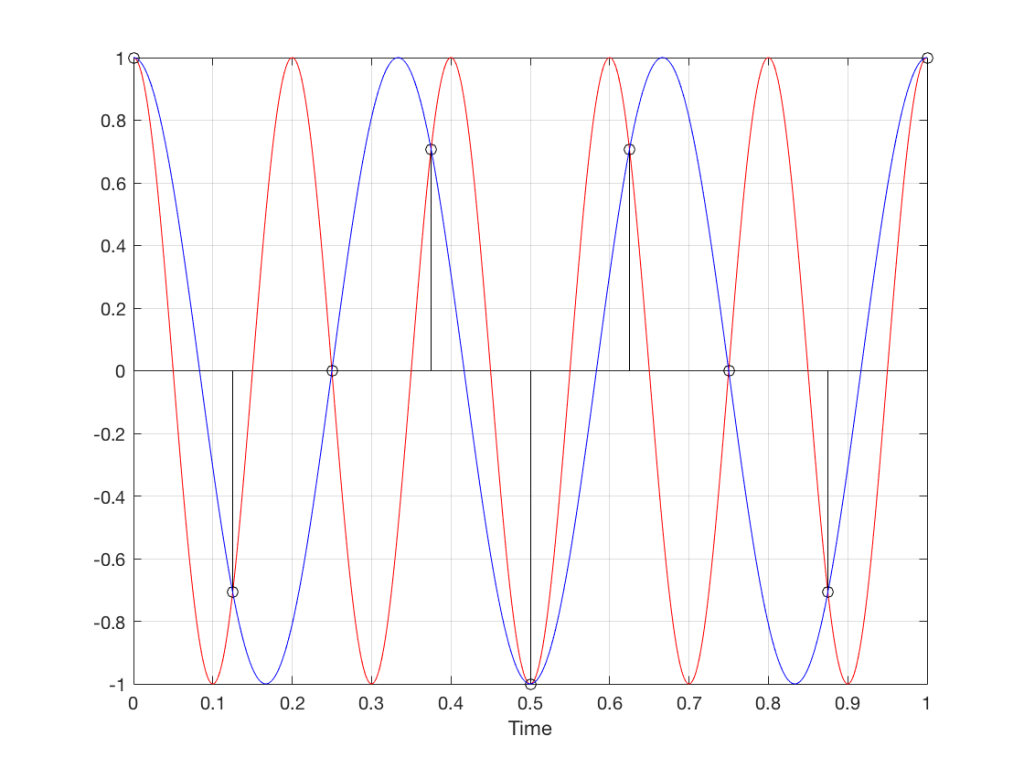Fig 12. The red signal and the black samples of it are the same as was shown in Figure 11. However, another frequency (the blue signal) also fits those samples. So, both the red signal and the blue signal exist in our system.

Moving up in frequency one more step, we get to the right-hand column in Figure 1, whose equivalent, including the aliased signal, are shown in Figure 13.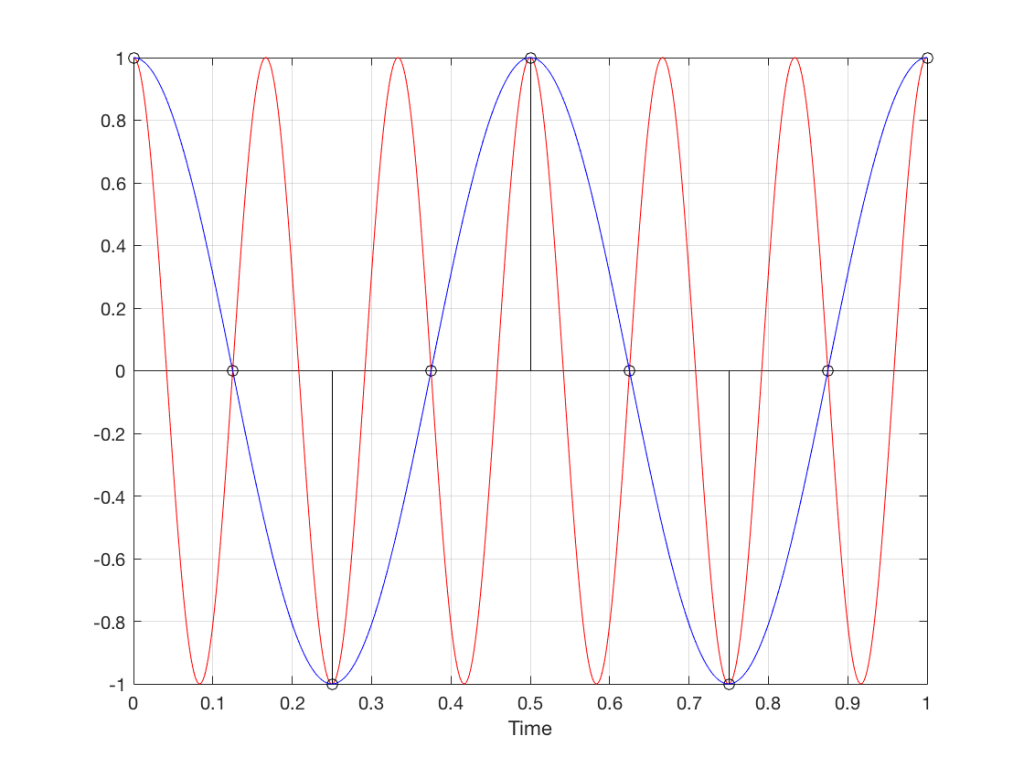Fig 13. A signal (the red curve) that has a frequency equivalent to 280º of rotation per sample, its samples (the black lollipops) and the aliased additional signal that results (the blue curve).

## Do I need to worry yet?

Hopefully, now, you can see that an LPCM system has a limit with respect to the maximum frequency that it can deal with appropriately. Specifically, the signal that you are trying to capture CANNOT exceed one-half of the sampling rate. So, if you are recording a CD, which has a sampling rate of 44,100 samples per second (or 44.1 kHz) then you CANNOT have any audio signals in that system that are higher than 22,050 Hz.

That limit is commonly known as the “Nyquist frequency“, named after Harry Nyquist – one of the persons who figured out that this limit exists.

In theory, this is always true. So, when someone did the recording destined for the CD, they made sure that the signal went through a low-pass filter that eliminated all signals above the Nyquist frequency.

In practice, however, there are many cases where aliasing occurs in digital audio systems because someone wasn’t paying enough attention to what was happening “under the hood” in the signal processing of an audio device. This will come up later.

## Two more details to remember…

There’s an easy way to predict the output of a system that’s suffering from aliasing if your input is sinusoidal (and therefore contains only one frequency). The frequency of the output signal will be the same distance from the Nyquist frequency as the frequency if the input signal. In other words, the Nyquist frequency is like a “mirror” that “reflects” the frequency of the input signal to another frequency below Nyquist.

This can be easily seen in the upper plot of Figure 14. The distance from the Input signal and the Nyquist is the same as the distance between the output signal and the Nyquist.

Also, since that Nyquist frequency acts as a mirror, then the Input and output signal’s frequencies will move in opposite directions (this point will help later).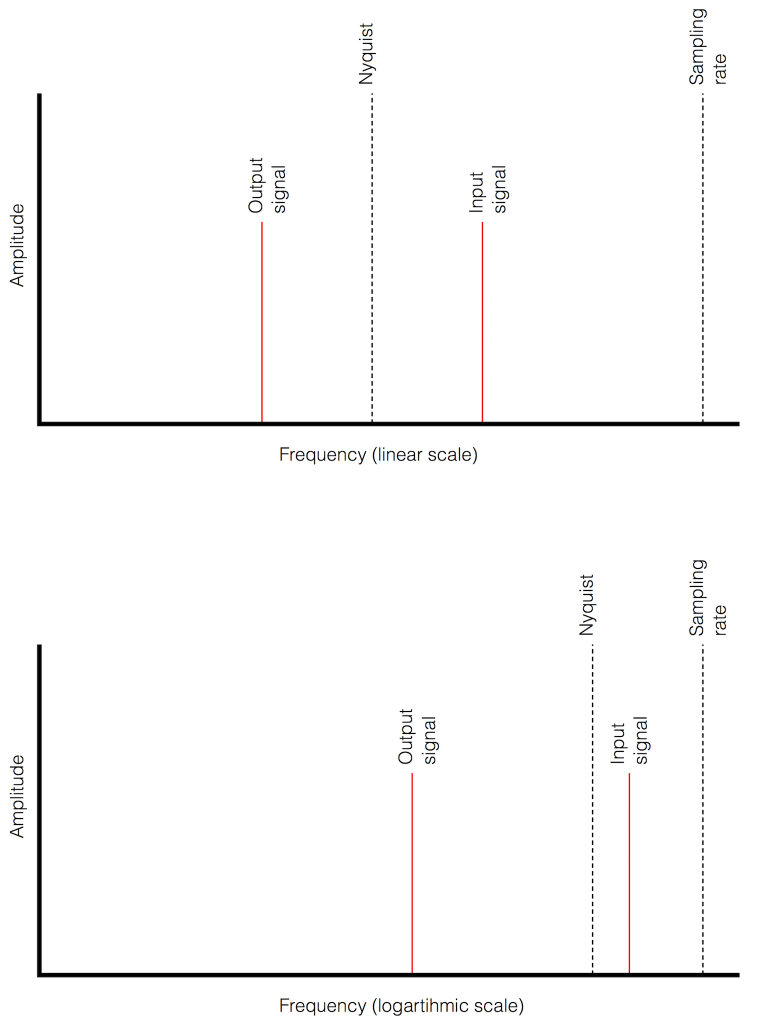Fig 14. Two plots showing the same information about an Input Signal above the Nyquist frequency and the output alias signal. Notice that, in the linear plot on top, it’s easier to see that the Nyquist frequency is the mirror point at the centre of the frequencies of the Input and Output signals.

Usually, frequency-domain plots are done on a logarithmic scale, because this is more intuitive for we humans who hear logarithmically. (For example, we hear two consecutive octaves on a piano as having the same “interval” or “width”. We don’t hear the width of the upper octave as being twice as wide, like a measurement system does. that’s why music notation does not get wider on the top, with a really tall treble clef.) This means that it’s not as obvious that the Nyquist frequency is in the centre of the frequencies of the input signal and its alias below Nyquist.

# Typical Errors in Digital Audio: Part 2 – Dither

Reminder: This is still just the lead-up to the real topic of this series. However, we have to get some basics out of the way first…

In the last posting, I talked about digital audio (more accurately, Linear Pulse Code Modulation or LPCM digital audio) is basically just a string of stored measurements of the electrical voltage that is analogous to the audio signal, which is a change in pressure over time…

For now, we’ll say that each measurement is rounded off to the nearest possible “tick” on the ruler that we’re using to measure the voltage. That rounding results in an error. However, (assuming that everything is working correctly) that error can never be bigger than 1/2 of a “step”. Therefore, in order to reduce the amount of error, we need to increase the number of ticks on the ruler.

Now we have to introduce a new word. If we really had a ruler, we could talk about whether the ticks are 1 mm apart – or 1/16″ – or whatever. We talk about the resolution of the ruler in terms of distance between ticks. However, if we are going to be more general, we can talk about the distance between two ticks being one “quantum” – a fancy word for the smallest step size on the ruler.

So, when you’re “rounding off to the nearest value” you are “quantising” the measurement (or “quantizing” it, if you live in Noah Webster’s country and therefore you harbor the belief that wordz should be spelled like they sound – and therefore the world needz more zees). This also means that the amount of error that you get as a result of that “rounding off” is called “quantisation error“.

In some explanations of this problem, you may read that this error is called “quantisation noise”. However, this isn’t always correct. This is because if something is “noise” then is is random, and therefore impossible to predict. However, that’s not strictly the case for quantisation error. If you know the signal, and you know the quantisation values, then you’ll be able to predict exactly what the error will be. So, although that error might sound like noise, technically speaking, it’s not. This can easily be seen in Figures 1 through 3 which demonstrate that the quantisation error causes a periodic, predictable error (and therefore harmonic distortion), not a random error (and therefore noise).

Sidebar: The reason people call it quantisation noise is that, if the signal is complicated (unlike a sine wave) and high in level relative to the quantisation levels – say a recording of Britney Spears, for example – then the distortion that is generated sounds “random-ish”, which causes people to just to the conclusion that it’s noise.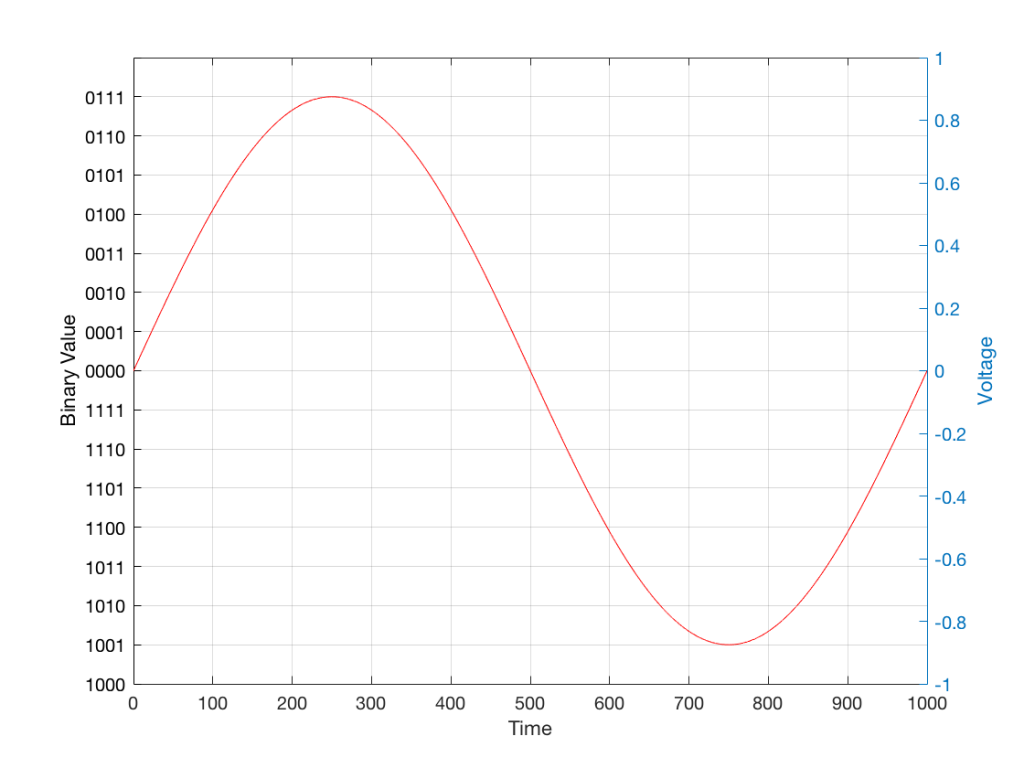Fig 1: The first cycle of a periodic signal (in this case, a sinusoidal waveform) that we are going to quantise using a 4-bit system (notice the 4 bits in the scale on the left).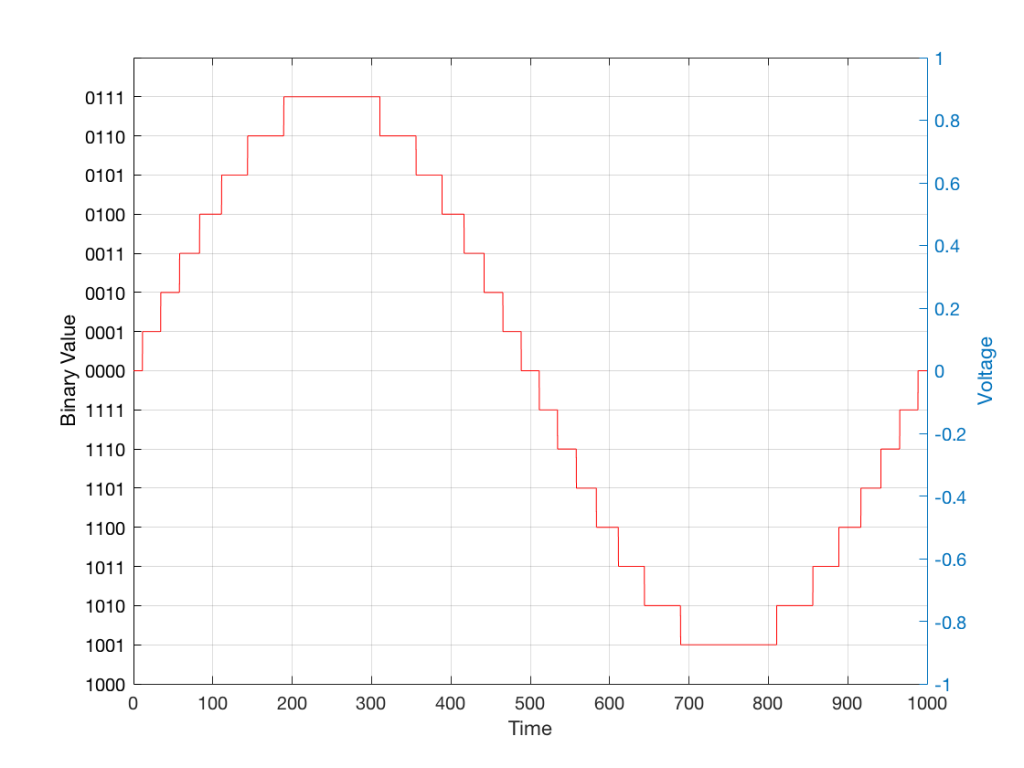Fig 2: The same waveform shown in Figure 1 after quantisation (rounding off) in a 4-bit world.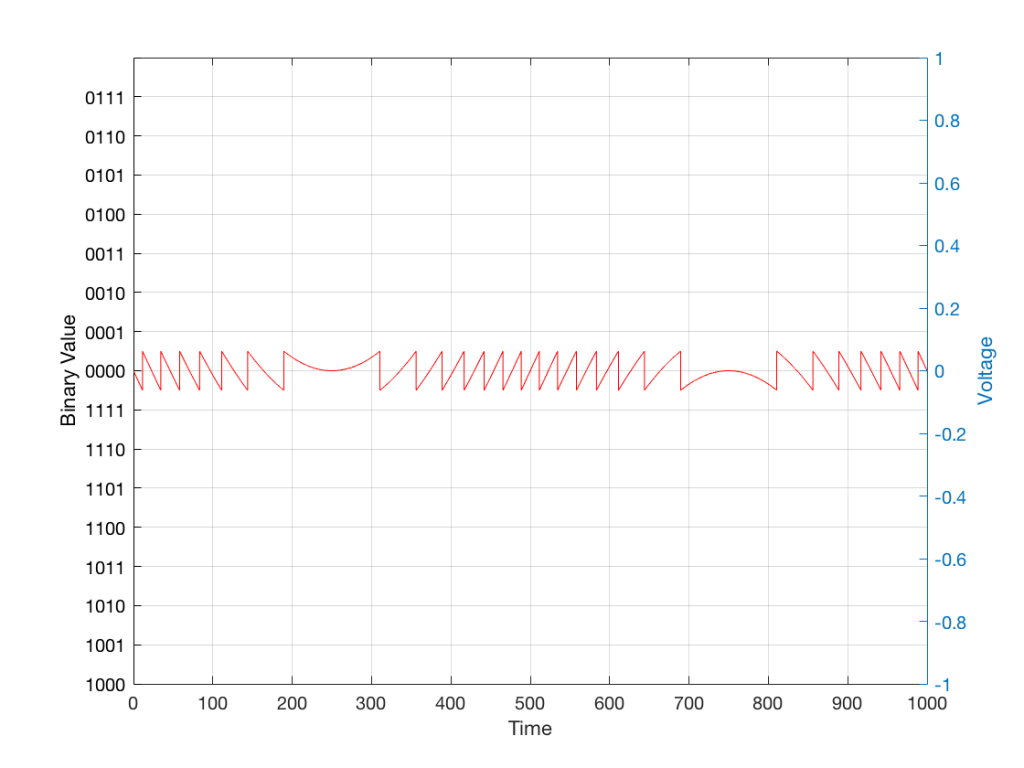Fig 3: The difference between Figure 2 and Figure 1. I made this by subtracting the original signal from the quantised version. This is the error in the quantised waveform – the quantisation error. Notice that it is not noise… it’s completely predictable and it will repeat with repetitions of the signal. Therefore the result of this is distortion, not noise…

Now, let’s talk about perception for a while… We humans are really good at detecting patterns – signals – in an otherwise noisy world. This is just as true with hearing as it is with vision. So, if you have a sound that exists in a truly random background noise, then you can focus on listening to the sound and ignore the noise. For example, if you (like me) are old enough to have used cassette tapes, then you can remember listening to songs with a high background noise (the “tape hiss”) – but it wasn’t too annoying because the hiss was independent of the music, and constant. However, if you, like me, have listened to Bob Marley’s live version of “No Woman No Cry” from the “Legend” album, then you, like me, would miss the the feedback in the PA system at that point in the song when the FoH engineer wasn’t paying enough attention… That noise (the howl of the feedback) is not noise – it’s a signal… Which makes it just as important as the song itself. (I could get into a long boring talk about John Cage at this point, but I’ll try to not get too distracted…)

The problem with the signal in Figure 2 is that the error (shown in Figure 3) is periodic – it’s a signal that demands attention. If the signal that I was sending into the quantisation system (in Figure 1) was a little more complicated than a sine wave – say a sine wave with an amplitude modulation – then the error would be easily “trackable” by anyone who was listening.

So, what we want to do is to quantise the signal (because we’re assuming that we can’t make a better “ruler”) but to make the error random – so it is changed from distortion to noise. We do this by adding noise to the signal before we quantise it. The result of this is that the error will be randomised, and will become independent of the original signal… So, instead of a modulating signal with modulated distortion, we get a modulated signal with constant noise – which is easier for us to ignore. (It has the added benefit of spreading the frequency content of the error over a wide frequency band, rather than being stuck on the harmonics of the original signal… but let’s not talk about that…)

## For example…

Let’s take a look at an example of this from an equivalent world – digital photography.

The photo in Figure 4 is a black and white photo – which actually means that it’s comprised of shades of gray ranging from black all the way to white. The photo has 272,640 individual pixels (because it’s 640 pixels wide and 426 pixels high). Each of those pixels is some shade of gray, but that shading does not have an infinite resolution. There are “only” 256 possible shades of gray available for each pixel.

So, each pixel has a number that can range from 0 (black) up to 255 (white).Fig 4: A photo of a building in Paris. Each pixel in this photo has one of 256 possible levels of gray – from white (255) down to black (0).

If we were to zoom in to the top left corner of the photo and look at the values of the 64 pixels there (an 8×8 pixel square), you’d see that they are:

86 86 90 88 87 87 90 91
86 88 90 90 89 87 90 91
88 89 91 90 89 89 90 94
88 90 91 93 90 90 93 94
89 93 94 94 91 93 94 96
90 93 94 95 94 91 95 96
93 94 97 95 94 95 96 97
93 94 97 97 96 94 97 97

What if we were to reduce the available resolution so that there were fewer shades of gray between white and black? We can take the photo in Figure 1 and round the value in each pixel to the new value. For example, Figure 5 shows an example of the same photo reduced to only 4 levels of gray.Fig 5: The same photo of the same building. Each pixel in this photo has one of 4 possible levels of gray – 255 (white), 170, 85 and 0 (black). Notice that some details are lost – like the smooth transitions in the clouds, or the stripes in the marble in the pillars.

Now, if we look at those same pixels in the upper left corner, we’d see that their values are

102 102 102 102 102 102 102 102
102 102 102 102 102 102 102 102
102 102 102 102 102 102 102 102
102 102 102 102 102 102 102 102
102 102 102 102 102 102 102 102
102 102 102 102 102 102 102 102
102 102 102 102 102 102 102 102
102 102 102 102 102 102 102 102

They’ve all been quantised to the nearest available level, which is 102. (Our possible values are restricted to 0, 51, 102, 154, 205, and 255).

So, we can see that, by quantising the gray levels from 256 possible values down to only 6, we lose details in the photo. This should not be a surprise… That loss of detail means that, for example, the gentle transition from lighter to darker gray in the sky in the original is “flattened” to a light spot in a darker background, with a jagged edge at the transition between the two. Also, the details of the wall pillars between the windows are lost.

If we take our original photo and add noise to it – so were adding a random value to the value of each pixel in the original photo (I won’t talk about the range of those random values…) it will look like Figure 6. This photo has all 256 possible values of gray – the same as in Figure 1.Fig 6: Noise. This “photo” has the same number of pixels (640 x 480) as the photo in Figure 4. We add this to the photo before asking the computer to reduce the number of “colours”.

If we then quantise Figure 6 using our 6 possible values of gray, we get Figure 7. Notice that, although we do not have more grays than in Figure 5, we can see things like the gradual shading in the sky and some details in the walls between the tall windows.Fig 7: The same photo of the same building in Figure 4. Each pixel in this photo ALSO only has one of 6 possible levels of gray – just like in Figure 5. However, this version is the result of quantising the original photo with the noise added before quantisation. The result is admittedly noisy – but we are able to see pattens in the noise that preserve some of the details that we lost in Figure 5.

That noise that we add to the original signal is called dither – because it is forcing the quantiser to be indecisive about which level to quantise to choose.

I should be clear here and say that dither does not eliminate quantisation error. The purpose of dither is to randomise the error, turning the quantisation error into noise instead of distortion. This makes it (among other things) independent of the signal that you’re listening to, so it’s easier for your brain to separate it from the music, and ignore it.

## Addendum: Binary basics and SNR

We normally write down our numbers using a “base 10” notation. So, when I write down 9374 – I mean
9 x 1000 + 3 x 100 + 7 x 10 + 4 x 1
or
9 x 103 + 3 x 102 + 7 x 101 + 4 x 100

We use base 10 notation – a system based on 10 digits (0 through 9) because we have 10 fingers.

If we only had 2 fingers, we would do things differently… We would only have 2 digits (0 and 1) and we would write down numbers like this:
11101

which would be the same as saying
1 x 16 + 1 x 8 + 1 x 4 + 0 x 2 + 1 x 1
or
1 x 24 + 1 x 23 + 1 x 22 + 0 x 21 + 1 x 20

The details of this are not important – but one small point is. If we’re using a base-10 system and we increase the number by one more digit – say, going from a 3-digit number to a 4-digit number, then we increase the possible number of values we can represent by a factor of 10. (in other words, there are 10 times as many possible values in the number XXXX than in XXX.)

If we’re using a base-2 system and we increase by one extra digit, we increase the number of possible values by a factor of 2. So XXXX has 2 times as many possible values as XXX.

Now, remember that the error that we generate when we quantise is no bigger than 1/2 of a quantisation step, regardless of the number of steps. So, if we double the number of steps (by adding an extra binary digit or bit to the value that we’re storing), then the signal can be twice as “far away” from the quantisation error.

This means that, by adding an extra bit to the stored value, we increase the potential signal-to-error ratio of our LPCM system by a factor of 2 – or 6.02 dB.

So, if we have a 16-bit LPCM signal, then a sine wave at the maximum level that it can be without clipping is about 6 dB/bit * 16 bits – 3 dB = 93 dB louder than the error. The reason we subtract the 3 dB from the value is that the error is +/- 0.5 of a quantisation step (normally called an “LSB” or “Least Significant Bit”).

Note as well that this calculation is just a rule of thumb. It is neither precise nor accurate, since the details of exactly what kind of error we have will have a minor effect on the actual number. However, it will be close enough.

# Typical Errors in Digital Audio: Part 1

## Introduction

Once upon a time, when I was a young whipper snapper, studying how to be a recording engineer (which is half of being a tonmeister) I had a textbook on sound recording. There were chapters in there on musical instruments, acoustics, microphones, mixing consoles, magnetic tape, and so on.. There was also a section on something called “digital audio” – but it was a portion of the chapter titled “Noise Reduction”.

Fast-forward a couple of years to 1983 and a new technology hit the market called “Compact Disc” (Here’s a fun fact for impressing people at your next dinner party: The “c” at the end of “disc” means it’s an optical medium. If it were magnetic, it would be a “disk”. So: Compact Disc, but Hard Disk.) Back then, the magazine advertisement read “Perfect Sound. Forever.” Then it hit the real world and the complaints started rolling in from people who believed that they knew things about audio. Some of these complaints were valid, and some were less so… Many of the ones that were valid no longer are, but it’s difficult to un-do a first impression.

Nowadays, it is very likely that almost-all-to-all of the music you listen to has been digital at some point in its life. Even if you’re listening to vinyl, it should not surprise you to know that the master version of the recording you’re hearing was probably stored on a hard disk or passed through a digital mixing console – or at least some of the tracks included some kind of digital processing (say, a guitar pedal or a reverb unit, for example). (I know, I know… There are exceptions. However, if you want to send me anti-digital hate mail you may not do it using a digital communication format such as e-mail. Use an analogue pen to write out your words on a piece of paper and send it to me by post. I look forward to receiving your analogue letters.)

Nowadays, a big part of my “day job” is to test (digital) audio systems to find out what’s wrong with them. So, I thought it would be interesting to do a series of postings that describe the typical kinds of errors that I look for (and find) when I’m digging down into the details.

In order to do this, I’m going to start by being a little redundant and describe the basics of how audio is converted from an analogue signal to a digital one – and hopefully address some of the misconceptions that are associated with this conversion process.

## A quick introduction to sound

At the simplest level, sound can be described as a small change in air pressure (or barometric pressure) over short periods of time. If you’d like to have a better and more edu-tain-y version of this statement with animations and pretty colours, you could take 10 minutes to watch this video, for example.

That change in pressure can be “captured” by using a microphone, that is (at the simplest level) a device that has a change in air pressure at its input and a change in electrical voltage at its output. Ignoring a lot of details, we could say that if you were to plot a measurement of the air pressure (at the input of the microphone) over time, and you were to compare it to a plot of the measurement of the voltage (at the output of the microphone) over time, you would see the same curve on the two graphs. This means that the change in voltage is analogous to the change in air pressure.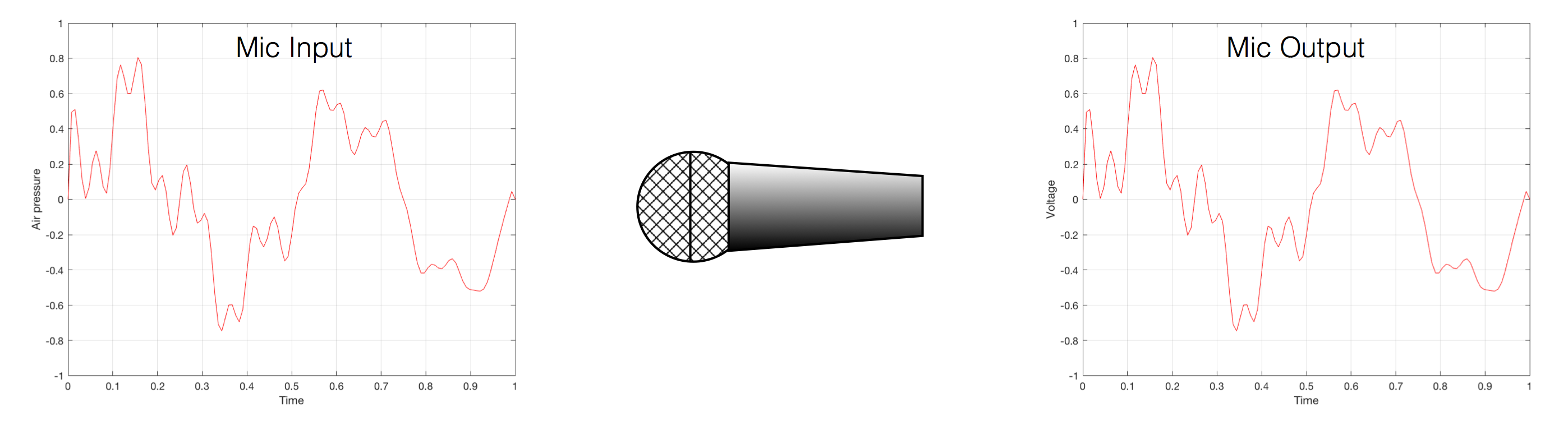Fig 1. Notice that (in theory, and ignoring a lot of things…) the change in air pressure over time at the input of the microphone is identical to the change in voltage over time at its output. Of course, this is not true in real life – microphones lie like a cheap rug…

At this point in the conversation, I’ll make a point to say that, in theory, we could “zoom in” on either of those two curves shown in Figure 1 and see more and more details. This is like looking at a map of Canada – it has lots of crinkly, jagged lines. If you zoom in and look at  the map of Newfoundland and Labrador, you’ll see that it has finer, crinkly, jagged lines. If you zoom in further, and stand where the water meets the shore in Trepassey and take a photo of your feet, you could copy it to draw a map of the line of where the water comes in around the rocks – and your toes – and you would wind up with even finer, crinkly, jagged lines… You could take this even further and get down to a microscopic or molecular level – but you get the idea… The point is that, in theory, both of the plots in Figure 1 have infinite resolution, both in time and in air pressure or voltage.

Now, let’s say that you wanted to take that microphone’s output and transmit it through a bunch of devices and wires that, in theory, all do nothing to the signal. Let’s say, for example, that you take the mic’s output, send it through a wire to a box that makes the signal twice as loud. Then take the output of that box and send it through a wire to another box that makes it half as loud. You take the output of that box and send it through a wire to a measuring device. What will you see? Unfortunately, none of the wires or boxes in the chain can be perfect, so you’ll probably see the signal plus something else which we’ll call the “error” in the system’s output. We can call it the error because, if we measure the input voltage and the output voltage at any one instant, we’ll probably see that they’re not identical. Since they should be identical, then the system must be making a mistake in transmitting the signal – so it makes errors…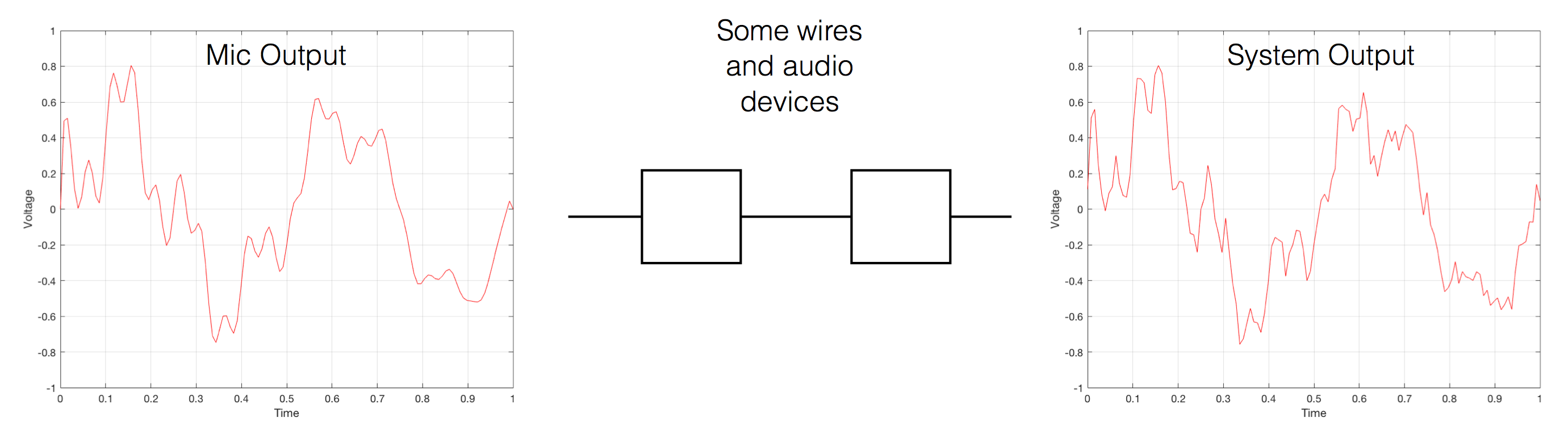Fig 2. If you send an audio signal through some wires and devices that (in theory) do nothing to the signal, you’ll find out that they add some extra stuff that you don’t want.

Pedantic Sidebar: Some people will call that error that the system adds to the signal “noise” – but I’m not going to call it that. This is because “noise” is a specific thing – noise is random – so if it’s not random, it’s not noise. Also, although the signal has been distorted (in that the output of the system is not identical to the input) I won’t call it “distortion” either, since distortion is a name that’s given to something that happens to the signal because the signal is there. (We would probably get at least some of the error out of our system even if we didn’t send any audio into it.) So, we could be slightly geeky and adequately vague and call the extra stuff “Distortion plus noise” but not “THD+N” – which stands for “Total Harmonic Distortion Plus Noise” – because not all kinds of distortion will produce a harmonic of the signal… but I’m getting ahead of myself…

So, we want to transmit (or store) the audio signal – but we want to reduce the noise caused by the transmission (or storage) system. One way to do this is to spend more money on your system. Use wires with better shielding, amplifiers with lower noise floors, bigger power supplies so that you don’t come close to their limits, run your magnetic tape twice as fast, and so on and so on. Or, you could convert the analogue signal (remember that it’s analogous to the change in air pressure over time) to one that is represented (and therefore transmitted or stored) digitally instead.

What does this mean?

## Conversion from analogue to digital and back (but skipping important details)

In the example above, we made a varying voltage that was analogous to the varying air pressure. If we wanted to store this, we could do it by varying the amount of magnetism on a wire or a coating on a tape, for example. Or we could cut a wiggly groove in a bit of vinyl that has a similar shape to the curve in the plots in Figure 1. Or, we could do something else: we could get a metronome (or a clock) and make a measurement of the voltage every time the metronome clicks, and write down the measurements.

For example, let’s zoom in on the first little bit of the signal in the plots in Figure 1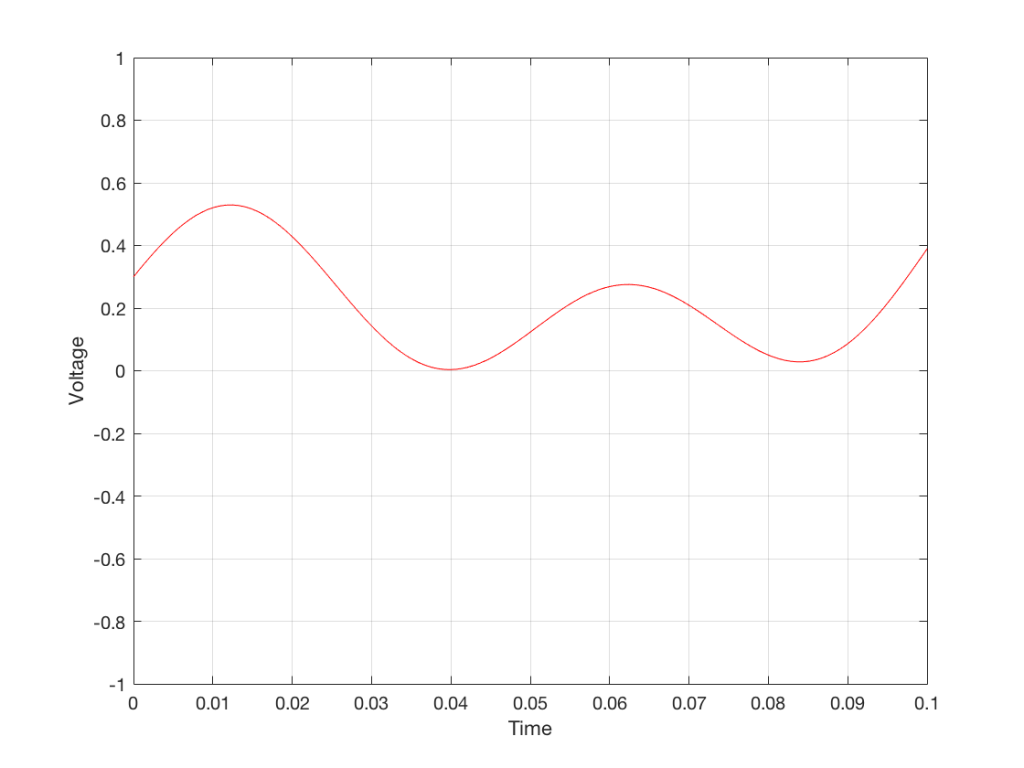Fig. 3 The same curve as was shown in Figure 1 – but zoomed in to the very beginning.

We’ll then put on a metronome and make a measurement of the voltage every time we hear the metronome click…

We can then keep the measurements (remembering how often we made them…) and write them down like this:

0.3000
0.4950
0.5089
0.3351
0.1116
0.0043
0.0678
0.2081
0.2754
0.2042
0.0730
0.0345
0.1775

We can store this series of numbers on a computer’s hard disk, for example. We can then come back tomorrow, and convert the measurements to voltages. First we read the measurements, and create the appropriate voltage…

We then make a “staircase” waveform by “holding” those voltages until the next value comes in.

All we need to do then is to use a low-pass filter to smooth out the hard edges of the staircase.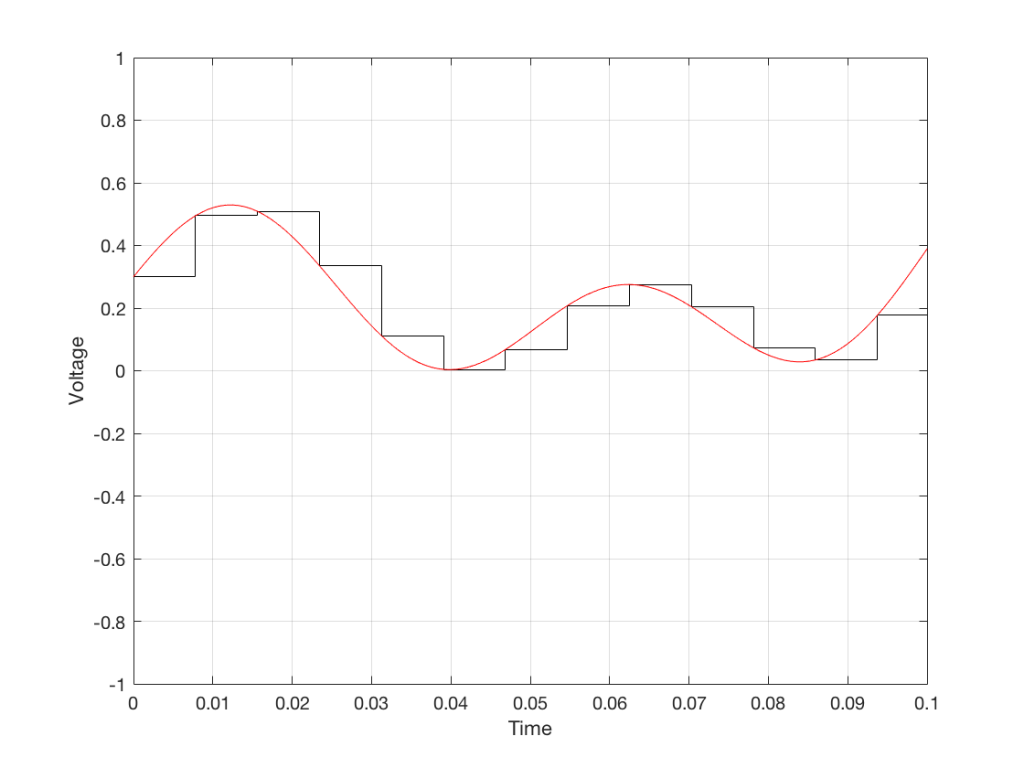Fig 7. When we smooth out the staircase, we get back the original signal (in red).

So, in this example, we’ve gone from an analogue signal (the red curve in Figure 3) to a digital signal (the series of numbers), and back to an analogue signal (the red curve in Figure 7).

In some ways, this is a bit like the way a movie works. When you watch a movie, you see a series of still photographs, probably taken at a rate of 24 pictures (or frames) per second. If you play those photos back at the same rate (24 fps or frames per second), you think you see movement. However, this is because your eyes and brain aren’t fast enough to see 24 individual photos per second – so you are fooled into thinking that things on the screen are moving.

However, digital audio is slightly different from film in two ways:

• The sound (equivalent to the movement in the film) is actually happening. It’s not a trick that relies on your ears and brain being too slow.
• If, when you were filming the movie, something were to happen between frames (say, the flash of a gunshot, for example) then it would never be caught on film. This is because the photos are discrete moments in time – and what happens between them is lost. However, if something were to make a very, very short sound between two samples (two measurements) in the digital audio signal – it would not be lost. This is because of something that happens at the beginning of the chain that I haven’t described… yet…

However, there are some “artefacts” (a fancy term for “weird errors”) that are present both in film and in digital audio that we should talk about.

The first is an error that happens when you mess around with the rate at which you take the measurements (called the “sampling rate”) or the photos (called the “frame rate”) – and, more importantly, when you need to worry about this. Let’s say that you make a film at 24 fps. If you play this back at a higher frame rate, then things will move very quickly (like old-fashioned baseball movies…). If you play them back at a lower frame rate, then things move in slow motion. So, for things to look “normal” you have to play the movie at the same rate that it was filmed. However, as longs no one is looking, you can transfer the movie as fast as you like. For example, if you wanted to copy the film, you could set up a movie camera so it was pointing at a movie screen and film the film. As long as the movie on the screen is running in sync with the camera, you can do this at any frame rate you like. But you’ll have to watch the copy at the same frame rate as the original film…

The second is an easy artefact to recognise. If you see a car accelerating from 0 to something fast on film, you’ll see the wheels of the car start to get faster and faster, then, as the car gets faster, the wheels slow down, stop, and then start going backwards… This does not happen in real life (unless you’re in a place lit with flashing lights like fluorescent bulbs or LED’s). I’ll do a posting explaining why this happens – but the thing to remember here is that the speed of the wheel rotation that you see on the film (the one that’s actually captured by the filming…) is not the real rotational speed of the wheel. However, those two rotational speeds are related to each other (and to the frame rate of the film). If you change the real rotational rate or the frame rate, you’ll change the rotational rate in the film. So, we call this effect “aliasing” because it’s a false version (an alias) of the real thing – but it’s always the same alias (assuming you repeat the conditions…) Digital audio can also suffer from aliasing, but in this case, you put in one frequency (which is actually the same as a rotational speed) and you get out another one. This is not the same as harmonic distortion, since the frequency that you get out is due to a relationship between the original frequency and the sampling rate, so the result is almost never a multiple of the input frequency.

## Some details that I left out…

One of the things I said above was something like “we measure the voltage and store the results” and the example I gave was a nice series of numbers that only had 4 digits after the decimal point. This statement has some implications that we need to discuss.

Let’s say that I have a thing that I need to measure. For example, Figure 8 shows a piece of metal, and I want to measure its width.

Using my ruler, I can see that this piece of metal is about 57 mm wide. However, if I were geeky (and I am) I would say that this is not precise enough – and therefore it’s not accurate. The problem is that my ruler is only graduated in millimetres. So, if I try to measure anything that is not exactly an integer number of mm long, I’ll either have to guess (and be wrong) or round the measurement to the nearest millimetre (and be wrong).

So, if I wanted you to make a piece of metal the same width as my piece of metal, and I used the ruler in Figure 8, we would probably wind up with metal pieces of two different widths. In order to make this better, we need a better ruler – like the one in Figure 9.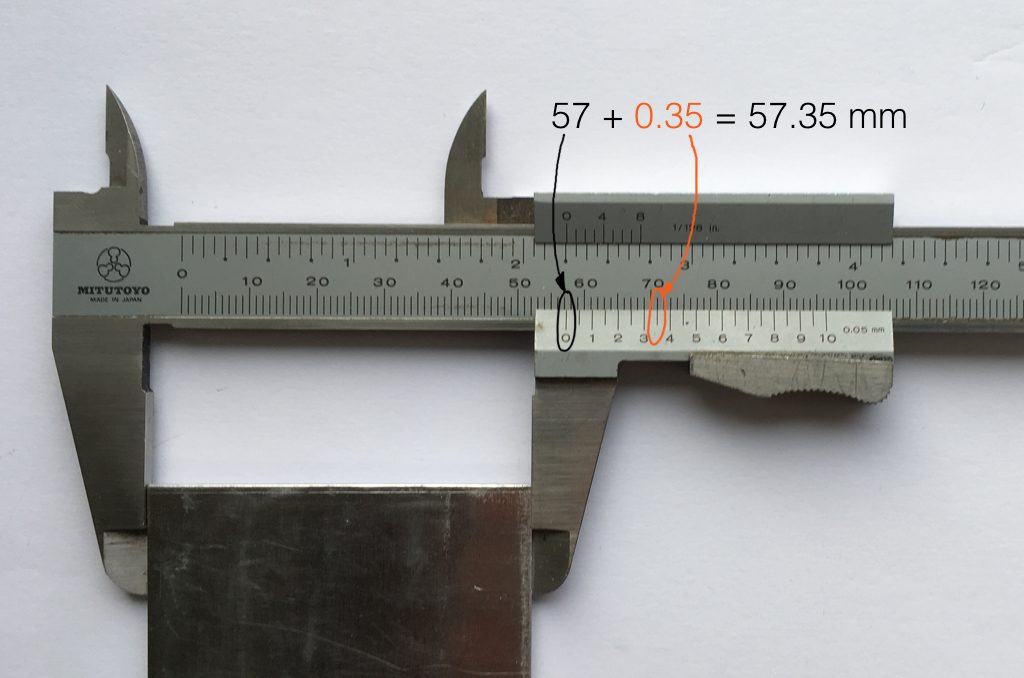Fig 9. The same piece of metal being measured with a vernier caliper. This gives us additional precision (down to 0.05 mm) so we can make a more accurate measurement.

Figure 9 shows a vernier caliper (a fancy type of ruler) being used to measure the same piece of metal. The caliper has a resolution of 0.05 mm instead of the 1 mm available on the ruler in Figure 8. So, we can make a much more accurate measurement of the metal because we have a measuring device with a higher precision.

The conversion of a digital audio signal is the same. As I said above, we measure the voltage of the electrical signal, and transmit (or store) the measurement. The question is: how accurate and precise is your measurement? As we saw above, this is (partly) determined by how many digits are in the number that you use when you “write down” the measurement.

Since the voltage measurements in digital audio are recorded in binary rather than decimal (we use 0 and 1 to write down the number instead of 0 up to 9) then we use Binary digITS – or “bits” instead of decimal digits (which are not called “dits”). The number of bits we have in the number that we write down (partly) determines the precision of the measurement of the voltage – and therefore (possibly), our accuracy…

Just like the example of the ruler in Figure 8, above, we have a limited resolution in our measurement. For example, if we had only 4 bits to work with then the waveform in 4 – the one we have to measure – would be measured with the “ruler” shown on the left side of Figure 10, below.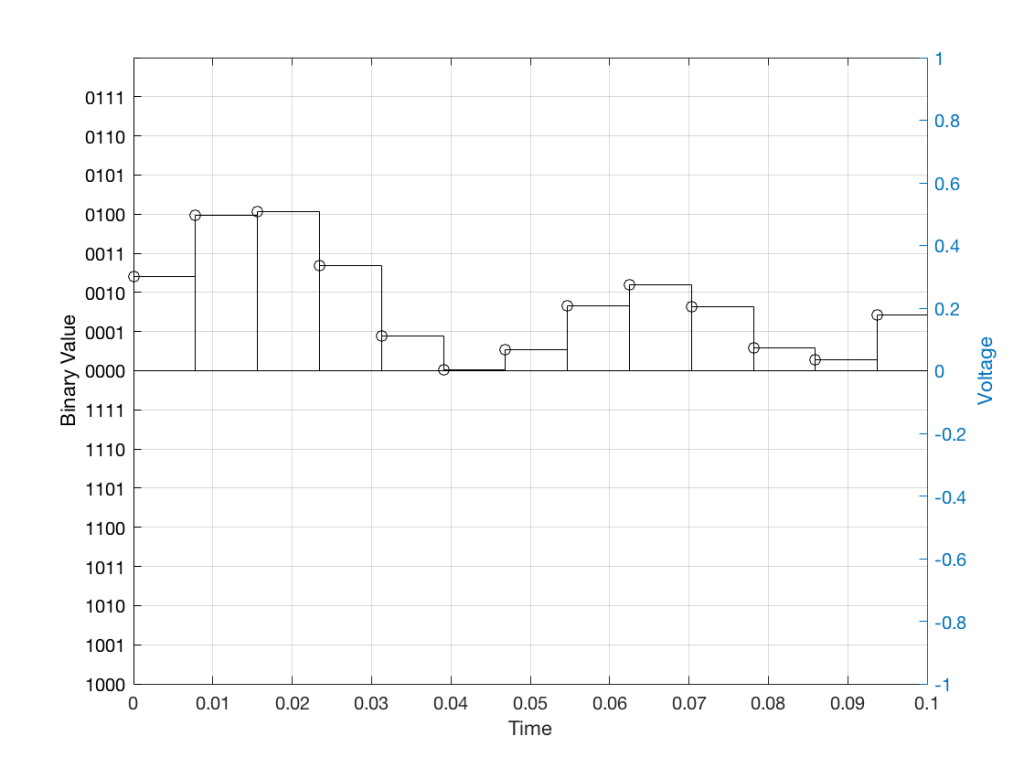Fig 10: The waveform from Figure 4 as a voltage (notice the Y-axis on the right). We have to measure these values using the ruler with the resolution shown on the Y-axis on the left.

When we do this, we have to round off the value to the nearest “tick” on our ruler, as shown in Figure 11.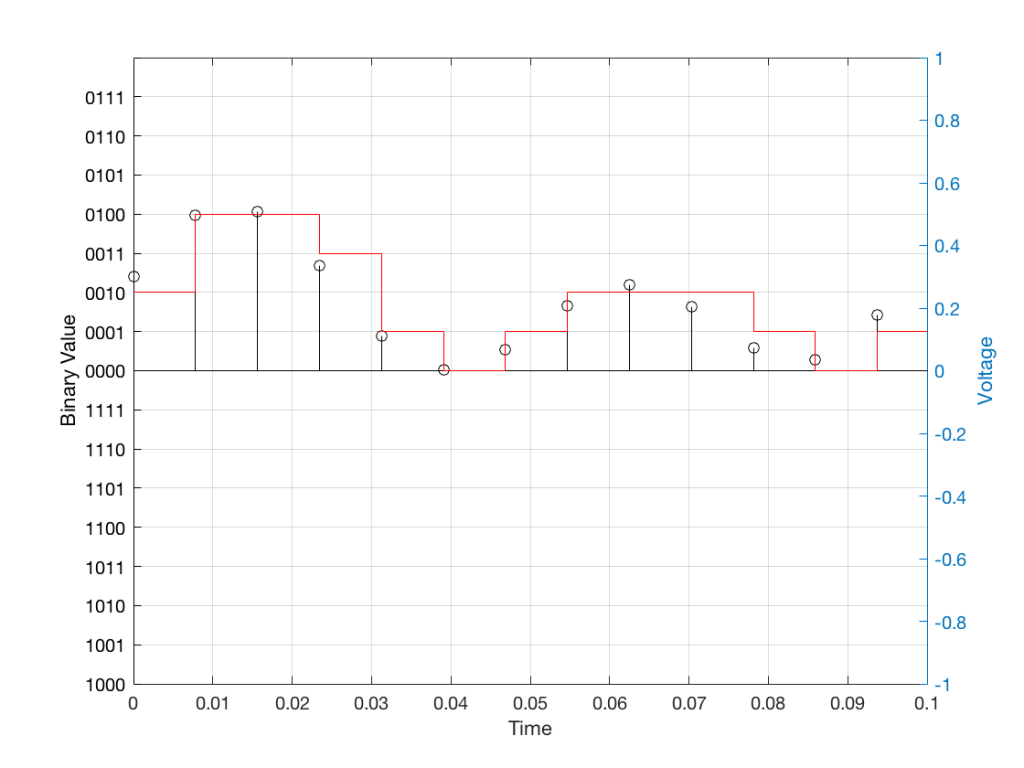Fig 11: The values from figure 10 (shown as the circles) rounded off to the nearest value on our 4-bit ruler (the red staircase).

Using this “ruler” which gives a write-down-able “quantity” to the measurement, we get the following values for the red staircase:

0010
0100
0100
0011
0001
0000
0001
0010
0010
0010
0001
0000
0001

When we “play these back” we get the staircase again, shown in Figure 12.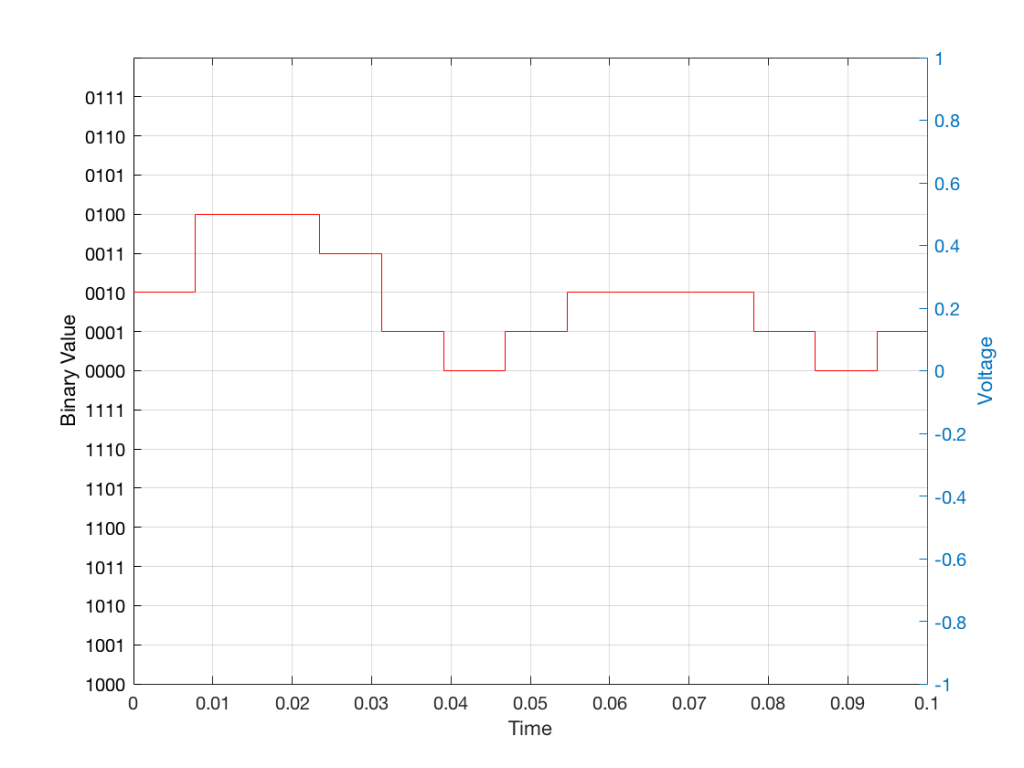Fig 12: The output of the measurements. Notice that all values sit exactly on one of the values for the “ruler” on the left Y-axis of the plot.

Of course, this means that, by rounding off the values, we have introduced an error in the system (just like the measurement in Figure 8 has a bigger error than the one in Figure 9). We can calculate this error if we just subtract the original signal from the output signal (in other words, Figure 12 minus Figure 10) to get Figure 13.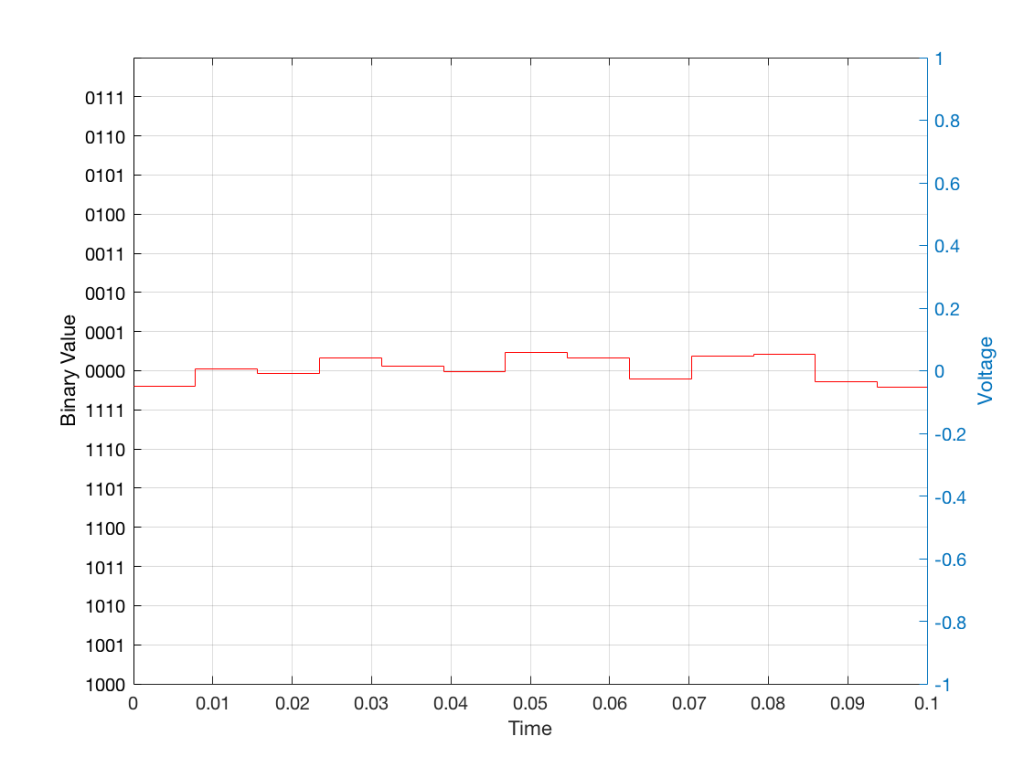Fig 13: The error that we produced due to the rounding off of the signal when we did the measurements. Notice that the error is always less than 0.5 of a “tick” of the ruler on the left Y-axis.

In order to improve our accuracy of the measurement, we have to increase the precision of the values. We can do this by adding an extra digit (or bit) to the number that we use to record the value.

If we were using decimal numbers (0-9) then adding an extra digit to the number would give us 10 times as many possibilities. (For example, if we were using 4 digits after the decimal in the example at the start of this posting, we have a total of 10,000 possible values – 0.0000 to 0.9999. If we add one more digit, we increase the resolution to 100,000 possible values – 0.00000 to 0.99999 ).

In binary, adding one extra digit gives us twice as many “ticks” on the ruler. So, using 4 bits gives us 16 possible values. Increasing to 5 bits gives us 32 possible values.

If you’re listening to a CD, then the individual measurements of each voltage – the “sample values” – are stored with 16 bits, which means that we have 65,536 possible values to pick from.

Remember that this means that we have more “ticks” on our ruler – but we don’t necessarily increase its range. So, for example, we’re still measuring a voltage from -1 V to 1 V – we just have more and more resolution to do that measurement with.

## Error #1

Finally! We get to the beginning of the point of the posting in the first place. My whole reason for starting this series of postings was to talk about errors in digital audio.

So, the first one to talk about is whether we have “bit matching” in a system where we expect to do so. For example, if you look at the S/P-DIF output of a good-old-fashioned CD player, do the sample values that are transmitted on that wire identical to the ones on the disc?

This is a fairly easy test to make (in theory). All you have to do is to record the digital signal on the S/P-DIF output of your CD player, subtract the original signal that’s on the disc (making sure that you have done your time alignment correctly). If you have anything other than nothing left over, then something went wrong somewhere.

If the result of this test is that you do NOT get nothing remaining, you cannot jump in head first and say that your S/P-DIF output is not working properly. For example, some sound cards have a sampling rate converter at their digital input. So, if you are capturing the CD player’s output using such a sound card on your computer, then perhaps the errors that you see are being produced by your sound card – and not your player.

### A little associated story

This was a method that I used to do the final testing of Wireless Power Link for B&O. I created a little software application that made a signal and sent it out digitally to a Wireless Power Link transmitter (which was running with a resolution of 24 bits – giving us 16,777,216 possible values). I then connected a Wireless Power Link receiver’s output to the same computer. The computer knew how much time it took the signal to get from its output, through the wireless transmission system, back to its input (about 5 ms). So, I took the “output” signal, delayed it by that amount, and then subtracted it from the “input” signal. I then made a detector that counted every bit (instead of every sample) that was incorrect.

The reason I was counting bit errors instead of sample errors was that we wanted to be able to diagnose problems if we found them. If you find out that “this sample is wrong” – you don’t necessarily know whether it was one or more bit errors that caused the problem. By counting bit errors, you have a little more information that can help you diagnose the source of problems when you find them.

Sidebar: since this test was running at 48 kHz and 24 bits with a 2-channel system, that means that there were 2,304,000 bits per second being checked every second

This test ran 24-hours a day continuously for over 11 days. In that time, we found 0 bit errors. That means that we got 0 errors in more than 2,189,721,600,000 bits, which was good.

Now, just before anyone gets excited: that test was run to find out whether the WPL system was able to deliver a bit-perfect output in the absence of any external disturbances. So, the transmitter and the receiver were not moved at any time during the test, and nothing was moved between them – and the result was that the system behaved perfectly.

# B&O Tech: Signal Mixing and Decomposition

#72 in a series of articles about the technology behind Bang & Olufsen loudspeakers

After a previous posting, someone posted this as part of a comment:

“What I’ve been asking myself for a long time: how does a single driver manage to produce two or more frequencies (or a frequency range) at the exact same time? For example a singer singing while the guitar plays in the background. Could you try to explain how this works?”

So, this posting is an attempt to answer that question.

Sound is a change of air pressure over time. That pressure is modulating on top of the day’s average barometric pressure – which is just a measurement of how closely the air particles around you are squeezed together. On a high-pressure day, the air is more densely packed – on a low pressure day, the air is less dense.

When you make a sound, you make slight variations in that pressure – so, for example, when a woofer moves out of a loudspeaker enclosure (a fancy name for “box”) then it pushes the air particles in front of it, and they’re squeezed together, resulting in a compression wave that radiates away from the loudspeaker. When the woofer pulls into the enclosure, it pulls the air particles apart, and you get a rarefaction wave instead. (You can see an animation of this at this posting.)

Let’s make a graph that shows a plot of the acoustic pressure changing over time. This is shown below in Figure 1. When this plot shows a positive number, it means that the air particles are being compressed more than normal. When it’s negative, then they’re being separated more than normal. Without getting into too many details, let’s just say that this is a low frequency. (If you want to get picky, then you’ll see that this is one cycle of a wave that takes 100 ms. Since there are 1000 ms in a second, then this must be a 10 Hertz signal, because 1000 / 100 = 10. That makes it a VERY low frequency by normal audio standards… )

Let’s also look at an example of a higher frequency, shown in Figure 2, below.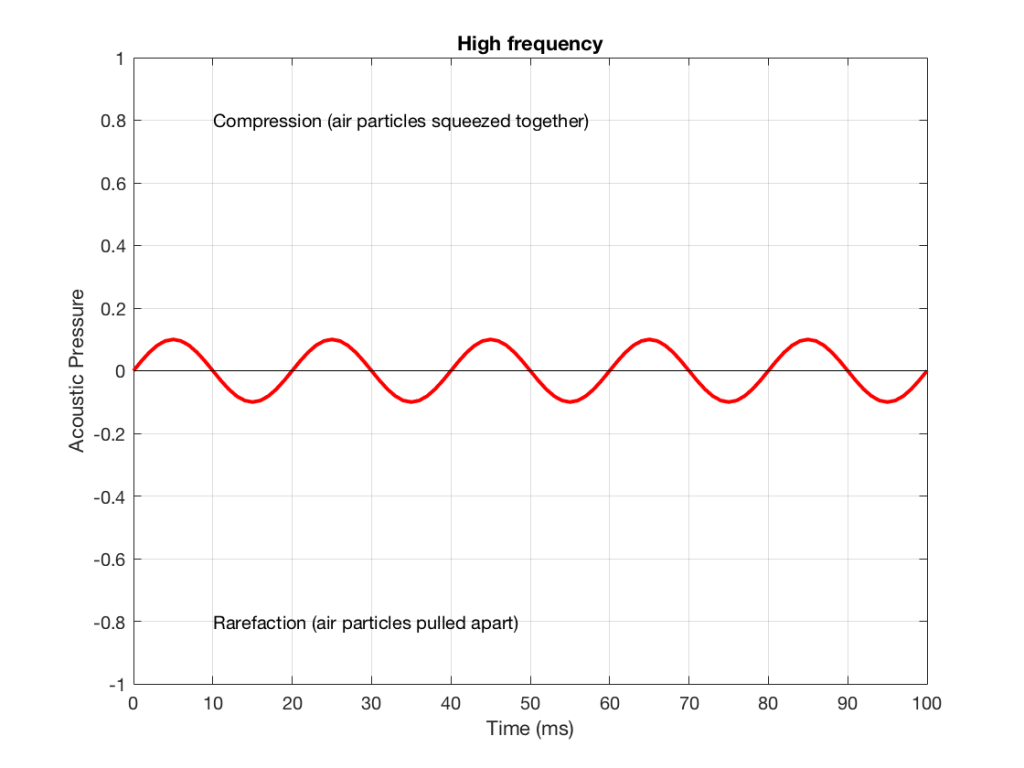Figure 2: A signal with a higher frequency and a lower amplitude than the one shown in Figure 1.

We can see that Figure 2 has a higher frequency signal, because it moves up and down more frequently. It has 5 cycles (5 ‘ups’ and ‘downs’) in the same amount of time that it took the wave in Figure 1 to have 1 cycle – therefore it is 5 times the frequency (and therefore, if you’re being picky, 50 Hz – which, by audio standards, is also a very low frequency, but this is just an example…)

Ignoring that ACTUAL frequencies that are plotted there, let’s pretend for a moment that the low frequency (Figure 1) came from a bass guitar and the higher frequency (Figure 2) came from a singer. If we took those two signals and put them into a mixing console, what does the result look like?

Well, we take the instantaneous value of the signal at one moment in time and add it to the instantaneous value of the other signal at the same time. Let’s do that.

Figure 3 shows the same signal as in Figure 1, but I’ve pointed out the values at two moments in time – at 25 ms and at 50 ms. So, for example, you can see there that, at 25 ms, the value is 0.5 – whatever that means…

Figure 4 shows the same signal as in Figure 2, but I’ve pointed out the values at two same moments in time – at 25 ms and at 50 ms. So, for example, you can see there that, at 25 ms, the value is 0.1 – whatever that means.

We take the value at 25 ms from each of the two signals (0.5 and 0.1) and add them together to get 0.6. This is the value of the signal at the output of the mixer at 25 ms. At 50 ms, the mixer’s output will have a value of 0 (because 0+0 = 0). This is shown graphically below for all of the values of both plots from 0 ms to 100 ms.

So, you can see in Figure 5 what the result will be. This signal contains both the low frequency, shown in Figure 1 and the higher frequency shown in Figure 2. If we send this combined signal to a loudspeaker, then both signals will get reproduced.

One interesting thing to note is that this mixing can also be done in the air. If a bass guitar and a singer are performing a song together, live, then the bass is pushing and pulling the molecules at the same time that the singer’s voice does. So, if at 25 ms, the bass pushes the molecules with a value of 0.5 (whatever that means) and the singer pushes the molecules with a value of 0.1, then your eardrum will be pushed in with a value of 0.6.  So, the summation of the pressure signals happens in the air, just like it does as voltages or voltage measurements in the mixing console.

## Splitting signals apart

Typically, however, a loudspeaker is comprised of more than one driver – for example, a woofer (for the low frequencies) and a tweeter (for the high frequencies). (Of course, some loudspeakers have more than two drivers, but we’re keeping things simple today…)

So, what we do there is to put the total signal, shown in Figure 5, and send it to two circuits that change how loud things are, depending on their frequency. One circuit is called a “low pass filter” because it allows low frequencies to pass through it unchanged, but it reduces the level of higher frequencies. The other circuit is called a “high pass filter” because it allows the high frequencies to pass through it unchanged, but it reduces the level of the lower frequencies. (we won’t talk about how those circuits do that in this posting…)

We can plot the two characteristics of these two circuits – an example of which is shown in Figure 6.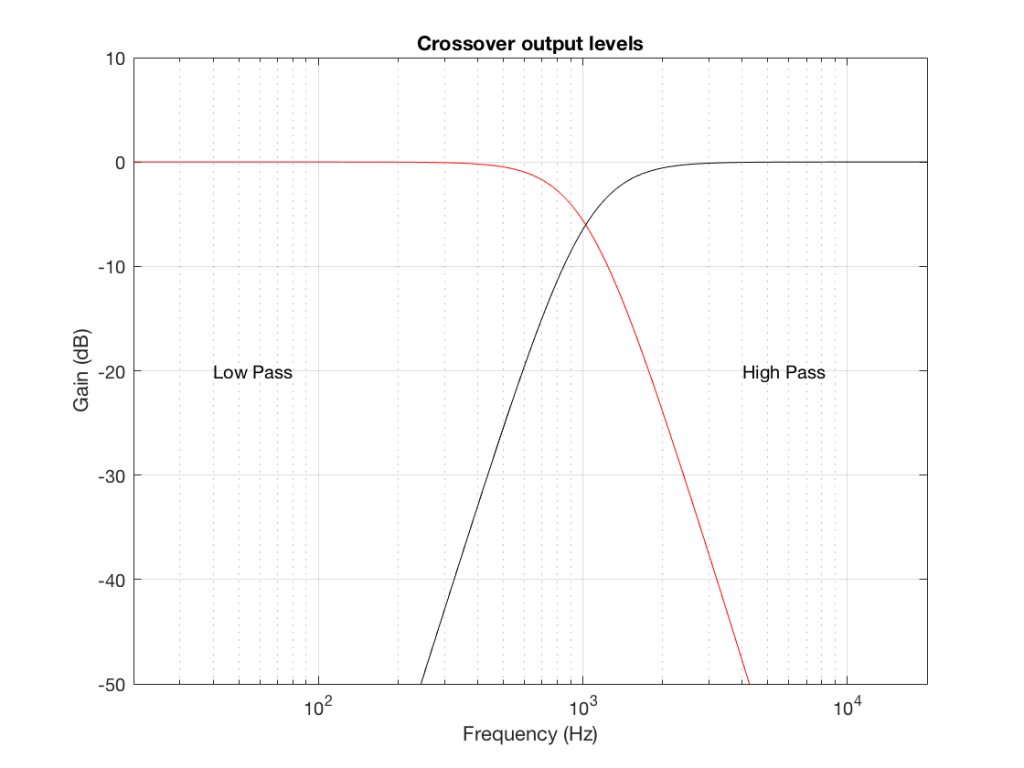Figure 6: An example of the gains applied to a signal by a low pass filter (in red) and a high pass filter (in black). Notice that, at one frequency, both filters have the same output level. That frequency is called the “crossover frequency” because it’s the frequency where the two filters’ responses cross over each other.

IF we send a signal like the one in Figure 5 to a crossover that happens to have a crossover frequency that is between the two frequencies it contains, then the signal will be split into two – one output containing mostly low-frequency components, and the other one containing mostly high-frequency components. Examples of these are shown below.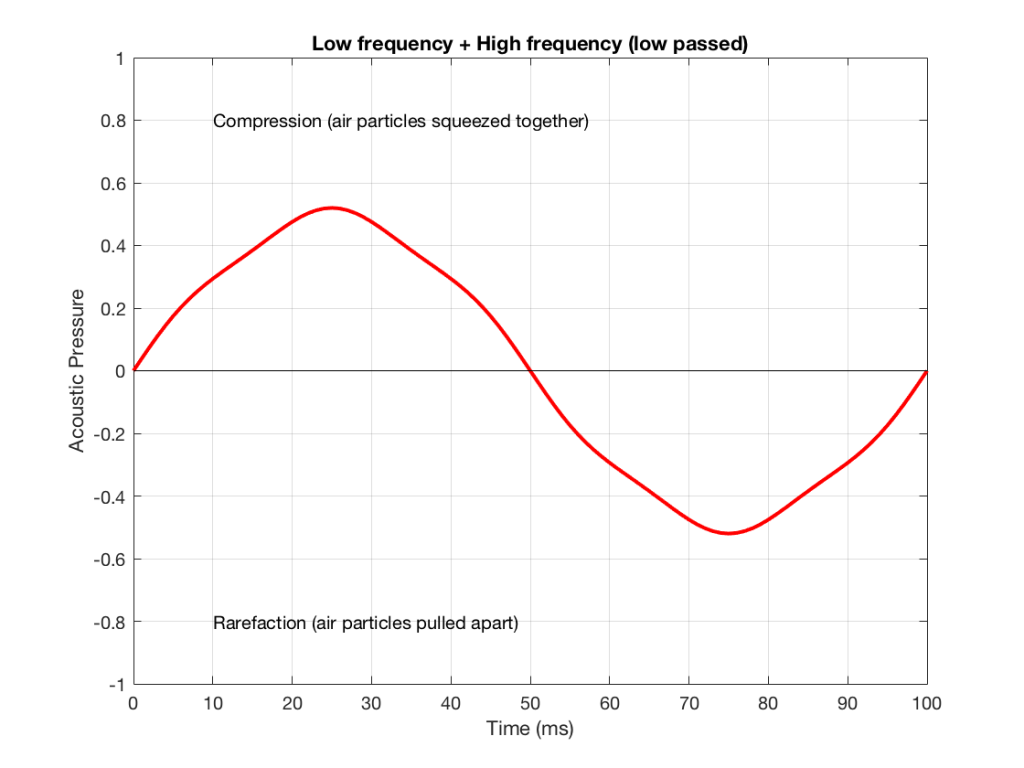Figure 7: An example of the output of the signal from Figure 5, sent though a low-pass filter. Notice that it looks a lot like Figure 1 (the low frequency component of the combined signal) – but there’s a little bit of Figure 2 (the high frequency component) left in there…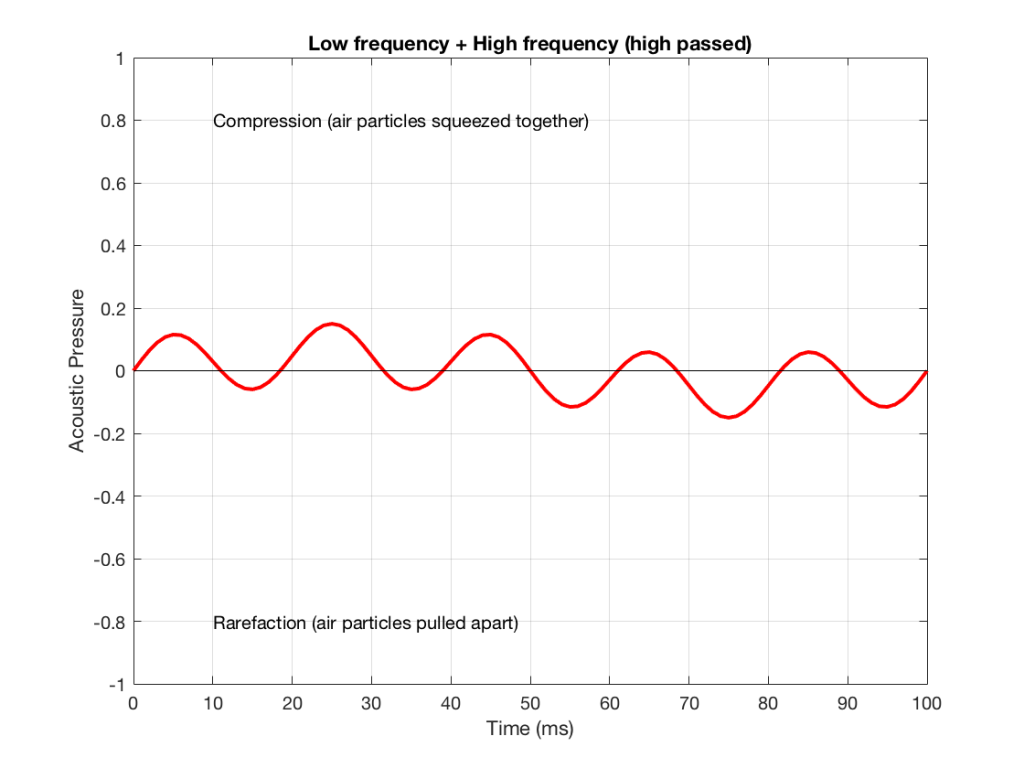Figure 8: An example of the output of the signal from Figure 5, sent though a high-pass filter. Notice that it looks a lot like Figure 2 (the high frequency component of the combined signal) – but there’s a little bit of Figure 1 (the low frequency component) left in there…

NB: Of course, everything I’ve shown here are just examples to make the concept intuitive. The crossover shown in Figure 6 would not work the way I’ve shown it in Figures 7 and 8 because the crossover frequency is too high compared to the 10 Hz and 50 Hz waves that I used in the example. So, please do not make comments talking about how I chose the wrong crossover frequency…